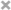# Xiuchuan Zhang

Personal Website

This is Xiuchuan's personal website.
I plan to post some of my current learning and review notes on it.
If you have any questions or suggestions, welcome to comment in my posts.

@TOC

## 基本概念

### 什么是期权？

• 期 -> 未来，权 -> 权利
• 期权 顾名思义 就是 追求 未来的权利
• 2015年 2月 9日，股票期权（option）入驻了我国证券市场。在中国有两种期权 50ETF 和 300 ETF。
• 期权也没有那么的复杂，和买保险差不多是一个意思。
• 例：张三 买了辆新车，并买入了一份一个月的保险（一个月的期权），如果 他的车在这个月内没有出问题，那么他就损失了这一部分保险费，如果这个月 车出了大毛病，那么他就有权利 去保险公司 所取赔偿（在期权内就是有权 不损失保险以外的金额）。
• 在期权里 期权费（保费）是不退的。

### 认购期权

• 又称 看涨期权
• 认购期权（call option），就是上面举的例子，购买认购期权就是 拥有 未来买入某个东西的权利。如果 这个东西在未来 价格 比你订的价格低，那么你就有权利不购买它，你就只损失这一笔 定金。
• 关键字：锁定 买入价

### 认沽期权

• 又称 看跌期权
• 认沽期权（put option），和认购相反，就是 在未来拥有卖出的权利。
• 关键字：锁定 卖出价

### 四种期权类型收益简单线性图

#### 买入看涨线性图（多头认购）

• 设定 我们 买入 期权价 500元的认购期权，定金100元。（支付100元 约定 一个月后 以500 元 价格 购买它）
• 一个月后 它的价格 变为 X。（若 X为 600，那么我不赚钱 $(600-500)-100$ ，若700 我赚一百 $(700-500)-100$， 若 400 我不履行合约 亏定金100 $(0-100)$)。
• 设定 因变量 Y 为 赚的钱， 就可以 画出：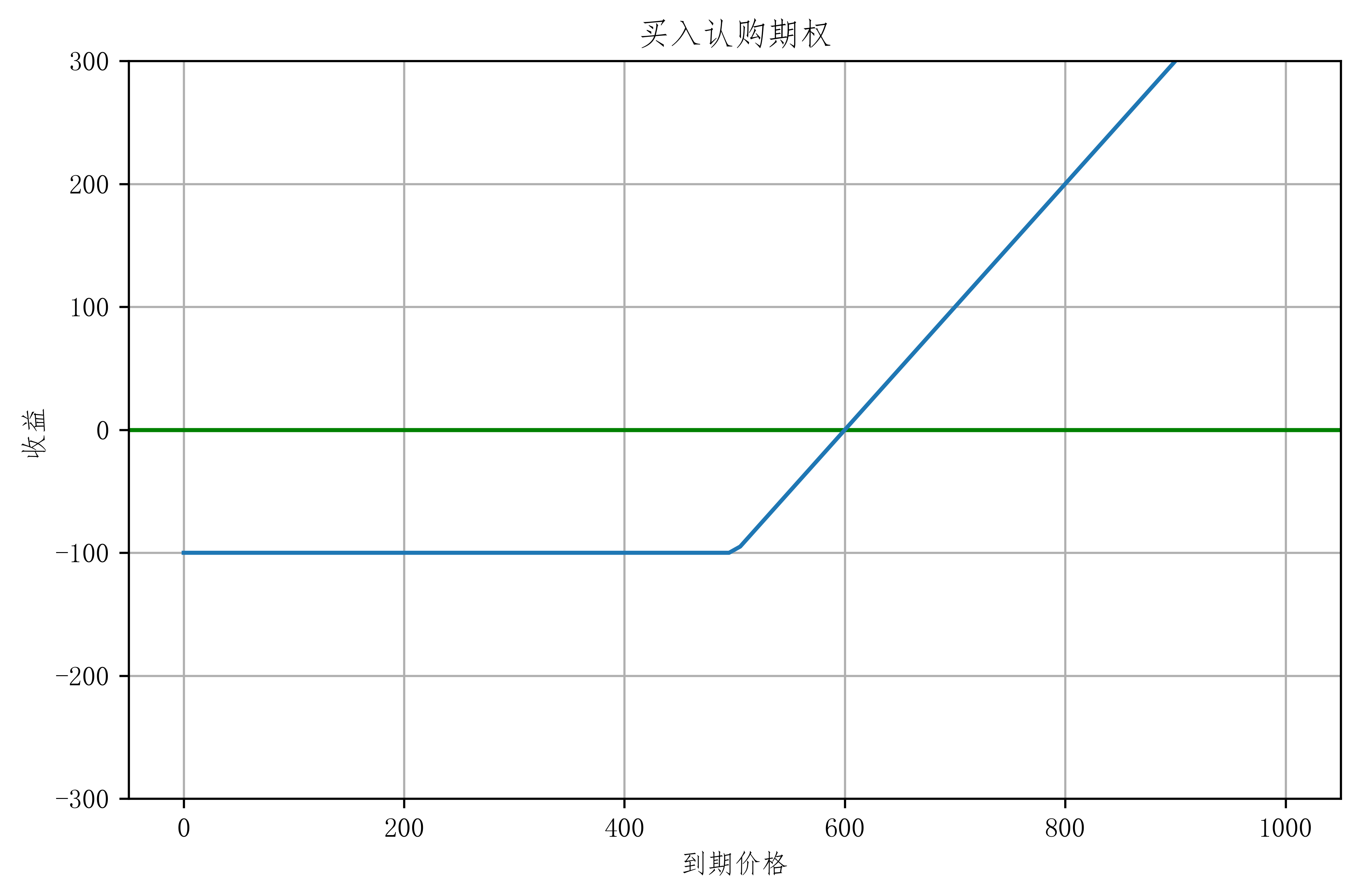#### 买入看跌线性图（多头认沽）

• 设定 我们 买入 期权价 500元的认沽期权（就是觉得一个月后 会跌），定金100元。（支付100元 约定 一个月后 以500 元 价格 卖出它）
• 一个月后 它的价格 变为 X。（若 X为 600，那么我不履行合约亏一百 $0-100$ ，若700 我不履行合约还亏一百 $0-100$， 若 400 我履行合约 但不赚钱 $(500-400)-100$)。
• 设定 因变量 Y 为 赚的钱， 就可以 画出：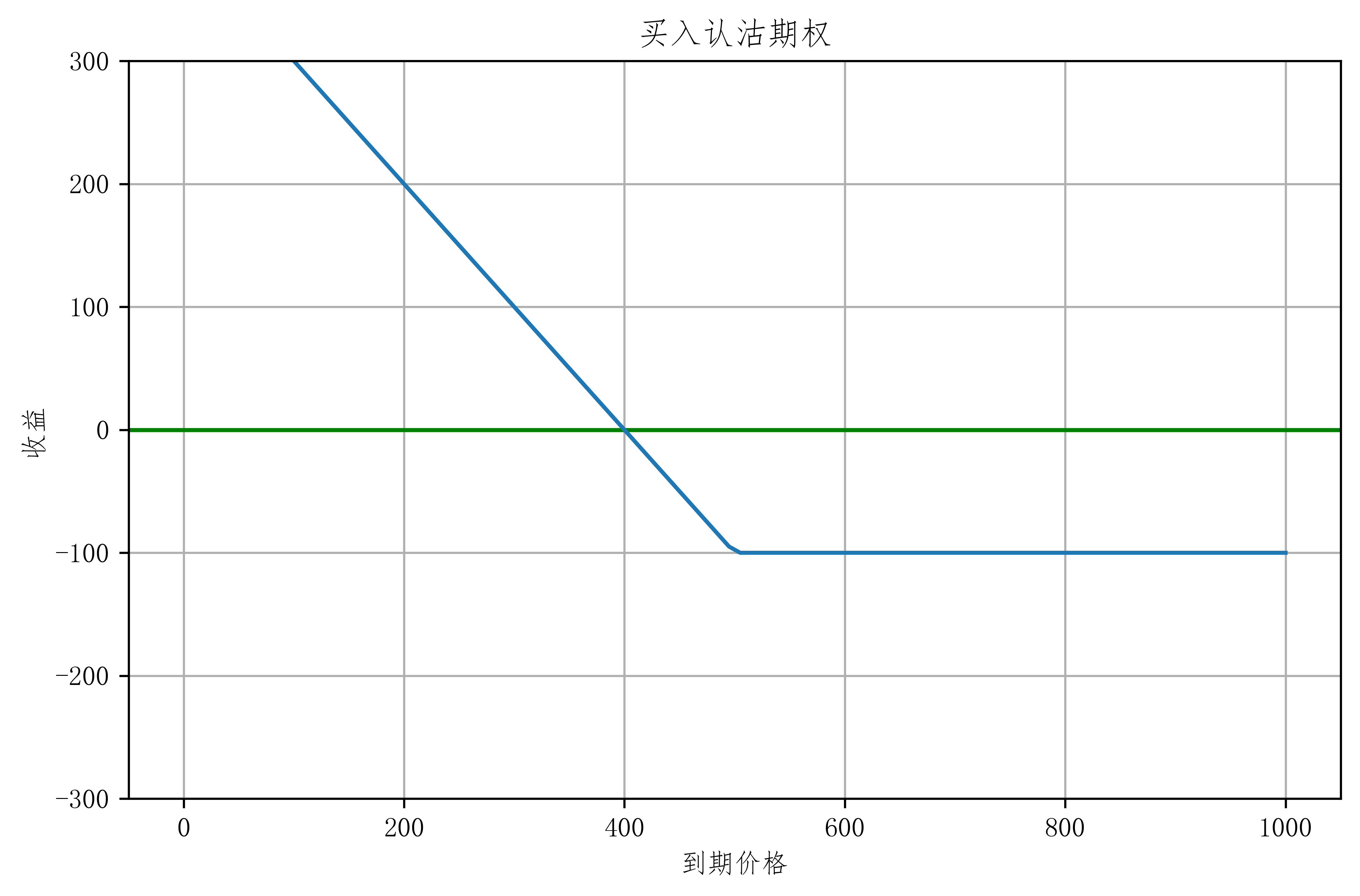• 那么 有了买入 那么 就有卖出 所以 我们 可以由买入 期权 变成卖出期权。
• 也就是 把 买入图 按x轴 倒一下 画出空头图

#### 卖出看涨线性图（空头认购）

• 设定 我们 卖出 期权价 500元的认购期权（就是觉得一个月后 不会涨），收入定金100元。（收入100元 约定 一个月后 以500 元 价格 卖出它），由于卖方没有权利毁约，所以一个月后必须卖出。
• 一个月后 它的价格 变为 X。
• 设定 因变量 Y 为 赚的钱， 就可以 画出：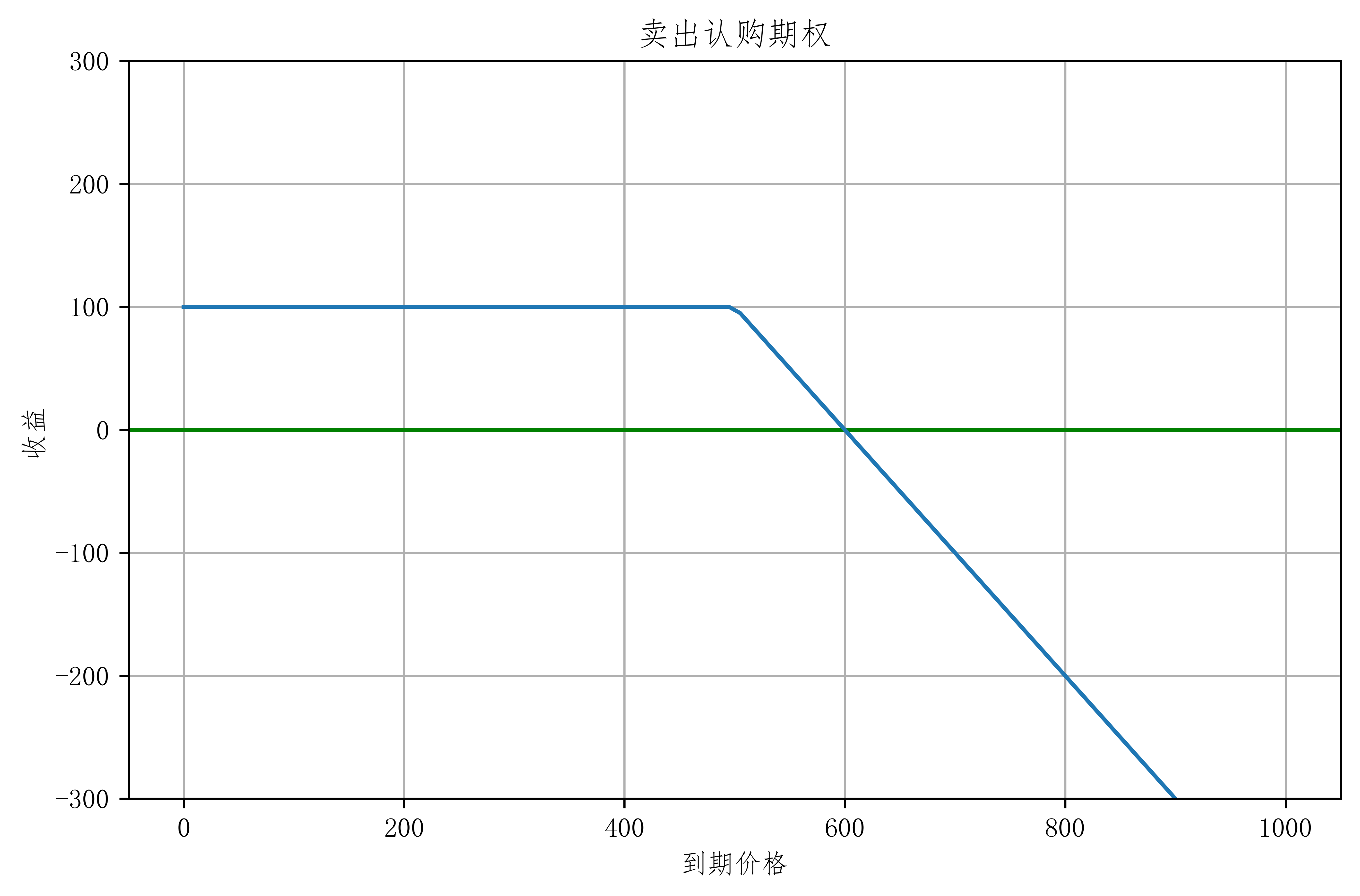#### 卖出看跌线性图（多头认沽）

• 设定 我们 卖出 期权价 500元的认沽期权（就是觉得一个月后 不会跌），收入定金100元。（收入100元 约定 一个月后 以500 元 价格 买入它），由于卖方没有权利毁约，所以一个月后必须卖出。
• 一个月后 它的价格 变为 X。
• 设定 因变量 Y 为 赚的钱， 就可以 画出：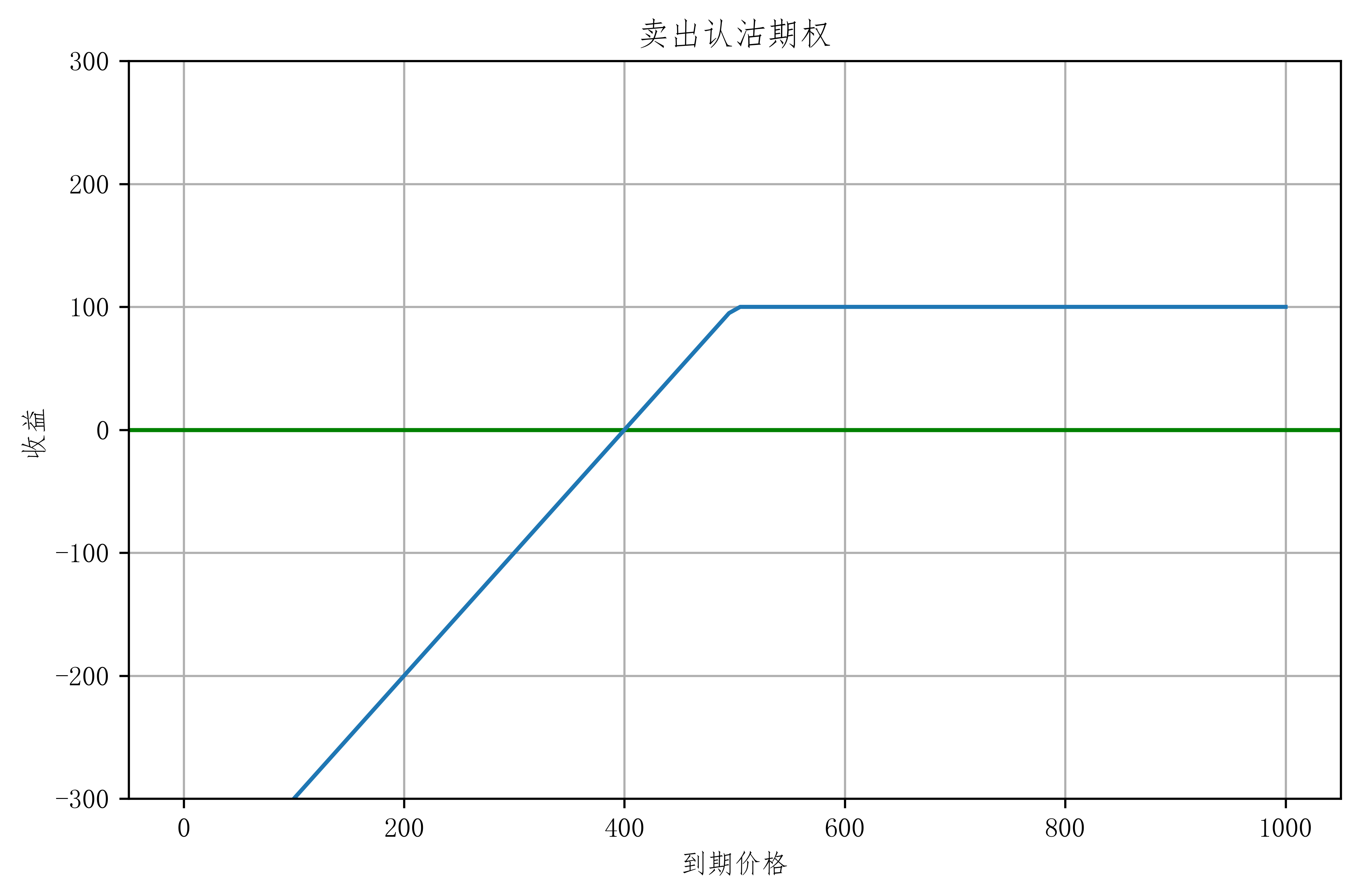#### 期权线性图

• 我们学会了 四种期权的简单构成 那么就可以画出所有的图 进行对比。
• 红色 为认购，绿色 为认沽
• 实线 为买入，虚线 为卖出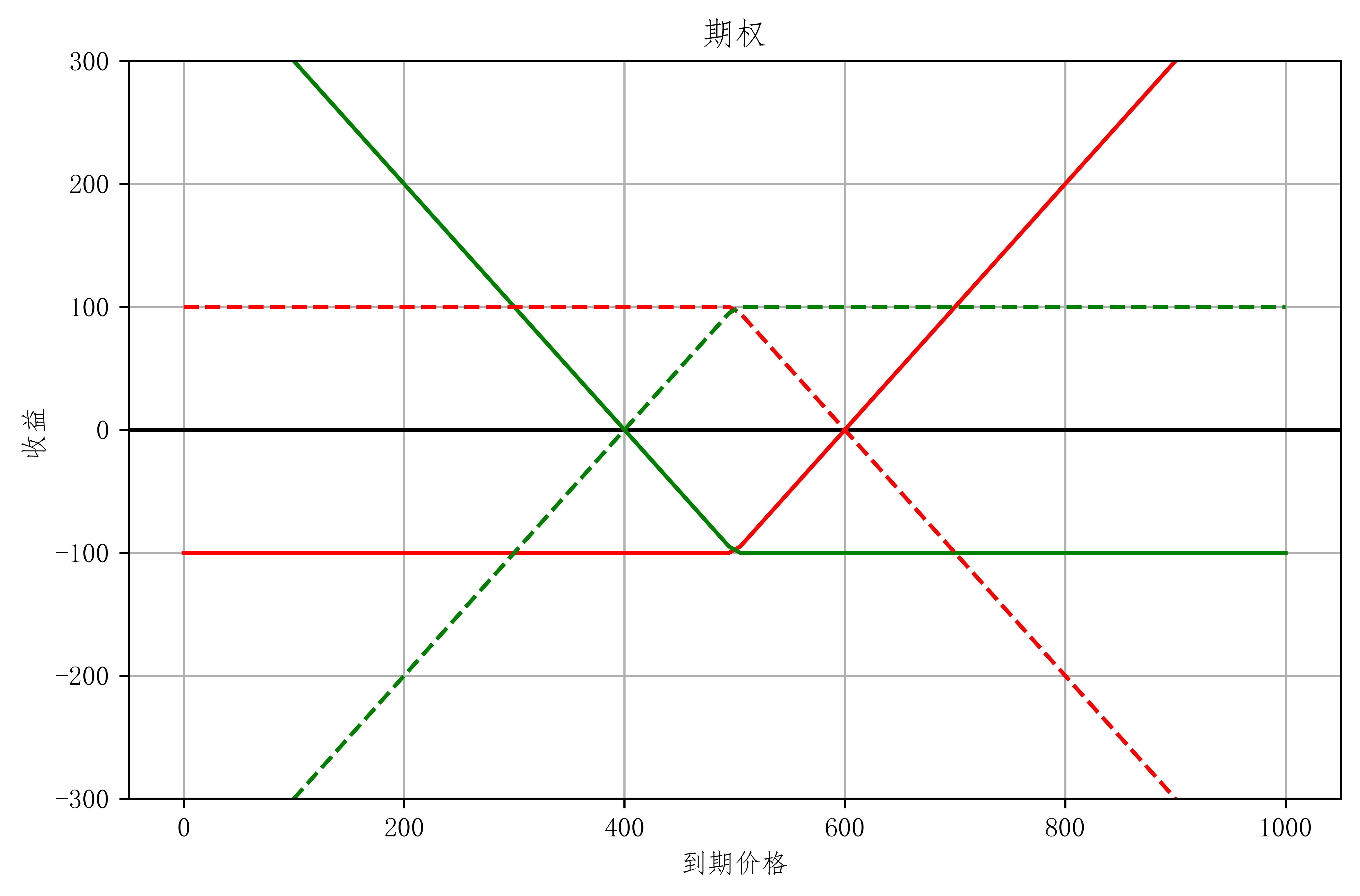• 在 图中 我们可以看到 这属于 零和博弈，有买入 那么 就有买出。在不考虑 税等因素情况下，买入的收益 就是卖出的亏损。
• 所以 期权是一种 即使 市场处于波动情况下 投资方 依然可以盈利的 金融产品。

• 补充说明（欧式期权 与 美式期权）：
• 在全球 有两种 期权 （或三种）
• 欧式期权：一种买方只能到期日当天行使权利 （中国使用的类型）
• 美式期权：一种买方可以在交易期间任何时间都可以行使权利
• (第三种)：在到期前指定一段时间可以行使权利
• 您 看到这 基本上了解了 期权的最基本的原理，现实交易肯定不可能这么的简单，那么接下来就 再 聊聊 现实中的 期权的时间价值 和 内在价值吧。

### 合约要素

#### 实值，虚值与平值

• 实值，虚值与平值 这三要素 理解起来很是简单：
• 实值：如果我现在行使权利 那我就赚了
• 虚值：如果我现在行使权利 那我就亏了 所以我不会行使权利
• 平值：如果我现在行使权利 不赚也不亏
• 我们用实例 来解释一下（我用的是 tushare，这是我的推荐码https://tushare.pro/register?reg=361123）：
import pandas as pd
import tushare as tus
pro = tus.pro_api()
# 获取 期权合约信息
opt_name = pro.opt_basic(exchange='SSE', fields='ts_code,name,exercise_type,list_date,delist_date')
# 选取 当月的合约
opt_name.query('list_date > "20200401" and delist_date < "20200529"')

ts_code name exercise_type list_date delist_date
925 10002475.SH 华夏上证50ETF期权2005认购3.00 欧式 20200420 20200527
926 10002476.SH 华夏上证50ETF期权2005认沽3.00 欧式 20200420 20200527
1019 10002471.SH 华夏上证50ETF期权2005认购2.95 欧式 20200408 20200527
1020 10002472.SH 华夏上证50ETF期权2005认沽2.95 欧式 20200408 20200527
1885 10002469.SH 华夏上证50ETF期权2005认购2.45 欧式 20200402 20200527
1886 10002470.SH 华夏上证50ETF期权2005认沽2.45 欧式 20200402 20200527
2314 10002473.SH 华泰柏瑞沪深300ETF期权2005认购4.20 欧式 20200408 20200527
2315 10002474.SH 华泰柏瑞沪深300ETF期权2005认沽4.20 欧式 20200408 20200527
• 介绍一下 上表信息。
• 50 ETF 和 300 ETF 是目前 中国唯二 的 两个 在证券交易所可交易的期权。
• 10002475.SH 为 在 tushare 的编号
• 华夏上证50ETF期权2005认购2.95 的意思是 投资者有权利以2.95元每份 买入 10000份 在2020年05月的 50ETF合约。也就是预期 在 5月 价格 会比 2.95元 高。
• 2020年4月17日 收盘价为 2.793 元。当天的价格如图：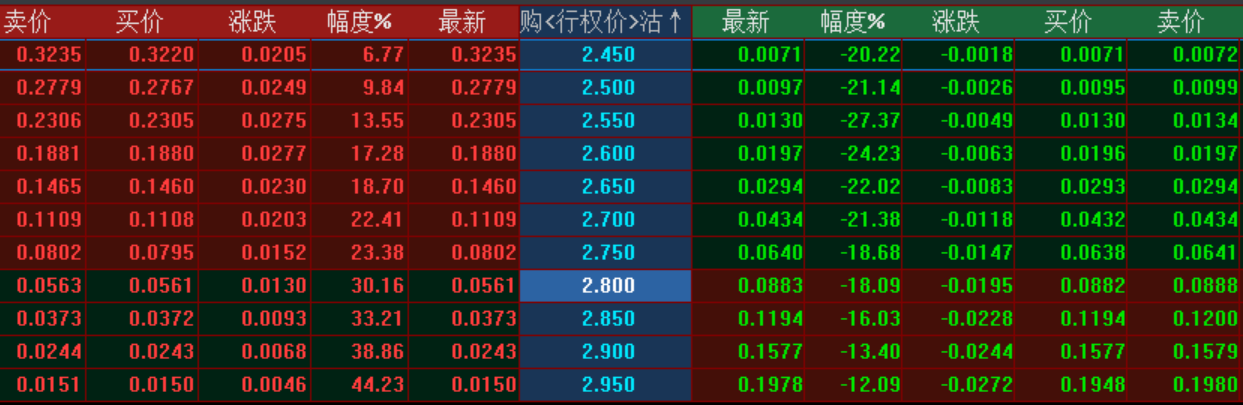• 若我们 在 2020年4月17日 以 0.0150元每份 买入 华夏上证50ETF期权2005认购2.95, 那么这时候 收盘价 对于行权价 2.95元 就为虚值，就是说 我现在就行使权利不合算，会亏本。
• 若我们 在 2020年4月17日 以 0.1978元 买入 华夏上证50ETF期权2005认沽2.95, 那么这时候 收盘价 对于行权价 2.95 就为实值，就是说 我现在就行使权利 会赚, 但是 我们还要考虑到买入的成本。

#### 内在价值与时间价值

• 我们通过上面的例子 可以算出 若 0.1978元 买入 2.95元的5月期货，那么对于 4月17日那天 收盘价2.793元 就直接行使权利的话 它的 内在价值为 $2.95 - 2.793 = 0.157$元。但是我们 又以 0.1978元购入，所以可以得出 它的时间价值为 $0.1978 - 0.157=0.0408$元。
• 在其他因素不变的话，距离到期时间越短，它的时间价值就越低。呈抛物线加速衰减。

• 接下来 看一下 认购期权 与 认沽期权 在 2020年04月的数据 (今日为4月19日 周日)
call295 = pro.opt_daily(ts_code='10002471.SH', start_date='20200408')
call295

ts_code trade_date exchange pre_settle pre_close open high low close settle vol amount oi
0 10002471.SH 20200417 SSE 0.0104 0.0104 0.0116 0.0225 0.0116 0.0150 0.0150 3.8711 6559168.0 51792.0
1 10002471.SH 20200416 SSE 0.0111 0.0111 0.0105 0.0117 0.0091 0.0104 0.0104 1.4148 1466280.0 39377.0
2 10002471.SH 20200415 SSE 0.0121 0.0121 0.0120 0.0170 0.0107 0.0111 0.0111 1.6649 2153384.0 34645.0
3 10002471.SH 20200414 SSE 0.0094 0.0094 0.0101 0.0123 0.0081 0.0121 0.0121 1.6154 1615393.0 28321.0
4 10002471.SH 20200413 SSE 0.0160 0.0160 0.0160 0.0160 0.0089 0.0094 0.0094 1.6560 1915005.0 23352.0
5 10002471.SH 20200410 SSE 0.0195 0.0195 0.0190 0.0216 0.0158 0.0160 0.0160 1.3566 2392633.0 17205.0
6 10002471.SH 20200409 SSE 0.0206 0.0206 0.0223 0.0228 0.0183 0.0195 0.0195 1.3723 2803389.0 12130.0
7 10002471.SH 20200408 SSE 0.0278 0.0278 0.0246 0.0266 0.0206 0.0206 0.0206 0.9021 2119503.0 5423.0
put295 = pro.opt_daily(ts_code='10002472.SH', start_date='20200408')
put295

ts_code trade_date exchange pre_settle pre_close open high low close settle vol amount oi
0 10002472.SH 20200417 SSE 0.2250 0.2250 0.2079 0.2079 0.1810 0.1978 0.1978 0.4036 7704138.0 7258.0
1 10002472.SH 20200416 SSE 0.2320 0.2320 0.2396 0.2399 0.2200 0.2250 0.2250 0.2473 5640617.0 6228.0
2 10002472.SH 20200415 SSE 0.2175 0.2173 0.2185 0.2320 0.2118 0.2320 0.2320 0.3116 6925738.0 5288.0
3 10002472.SH 20200414 SSE 0.2482 0.2482 0.2382 0.2460 0.2173 0.2173 0.2175 0.1450 3281409.0 3741.0
4 10002472.SH 20200413 SSE 0.2421 0.2407 0.2475 0.2529 0.2396 0.2482 0.2482 0.1281 3179785.0 2868.0
5 10002472.SH 20200410 SSE 0.2419 0.2414 0.2415 0.2483 0.2135 0.2407 0.2421 0.1260 2941148.0 1855.0
6 10002472.SH 20200409 SSE 0.2496 0.2496 0.2421 0.2437 0.2345 0.2414 0.2419 0.0572 1370615.0 1278.0
7 10002472.SH 20200408 SSE 0.2433 0.2433 0.2481 0.2569 0.2406 0.2496 0.2496 0.1524 3806835.0 1044.0
• 可以看到 由于认购期权 为虚值 购入的价格很低。这是因为 购买 虚值的期权 就像赌博，以小博大。例如，我在 04月17日时 购买了 一亿份 华夏上证50ETF期权2005认购2.95 花费 150万元 定金。若在5月27日时，价格涨到了3.05元 每份，那么我就相当于 赚了一千万元。若是没有比 2.95元高，那么我就损失 150万。在虚值交易中，这和杠杆类似。

## 期权的定义

• 上篇博客介绍了基本的概念。这次就来更深入的理解。
• 先回顾一下各个术语的定义。

• 权利的买方：期权多头
• 权利的卖方：期权空头
• 权利是未来买资产：认购期权，看涨期权
• 权利是未来卖资产：认沽期权，看跌期权
• 标的资产：买的是什么资产 和 多少数量
• 行权价：有权利买卖的价格
• 到期日：合约未来到期的日期

### 对比 期权与期货

• 期货 （Futures）

• 对比 期权与期货多头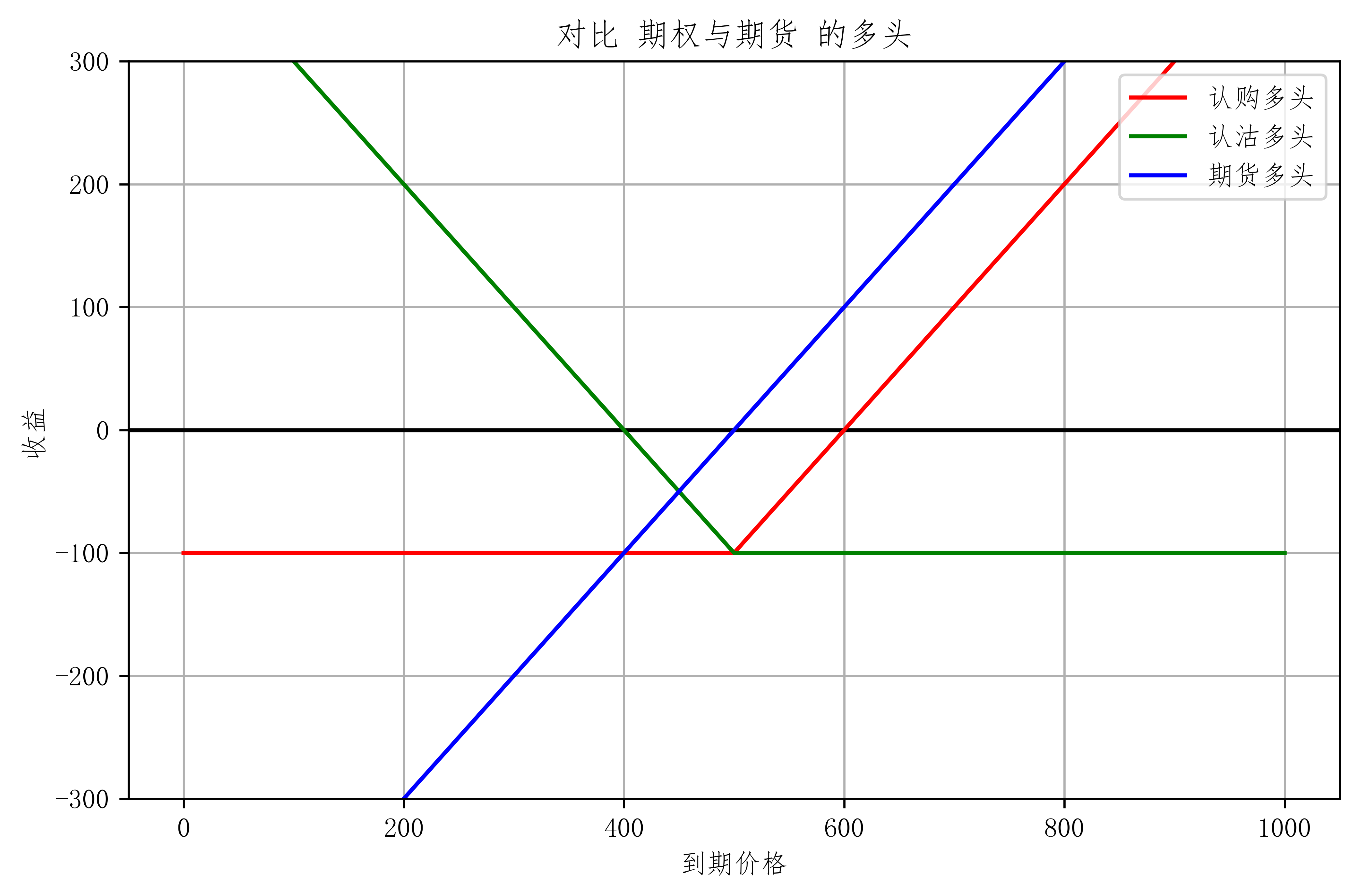• 由此可以看出，买入期权 主要是买入它的波动率，只要波动率越大，那么就越容易赚钱。而期货不同，它没有对波动有特别大的偏好，波动越大，代表风险越大。

• 对比 期权与期货空头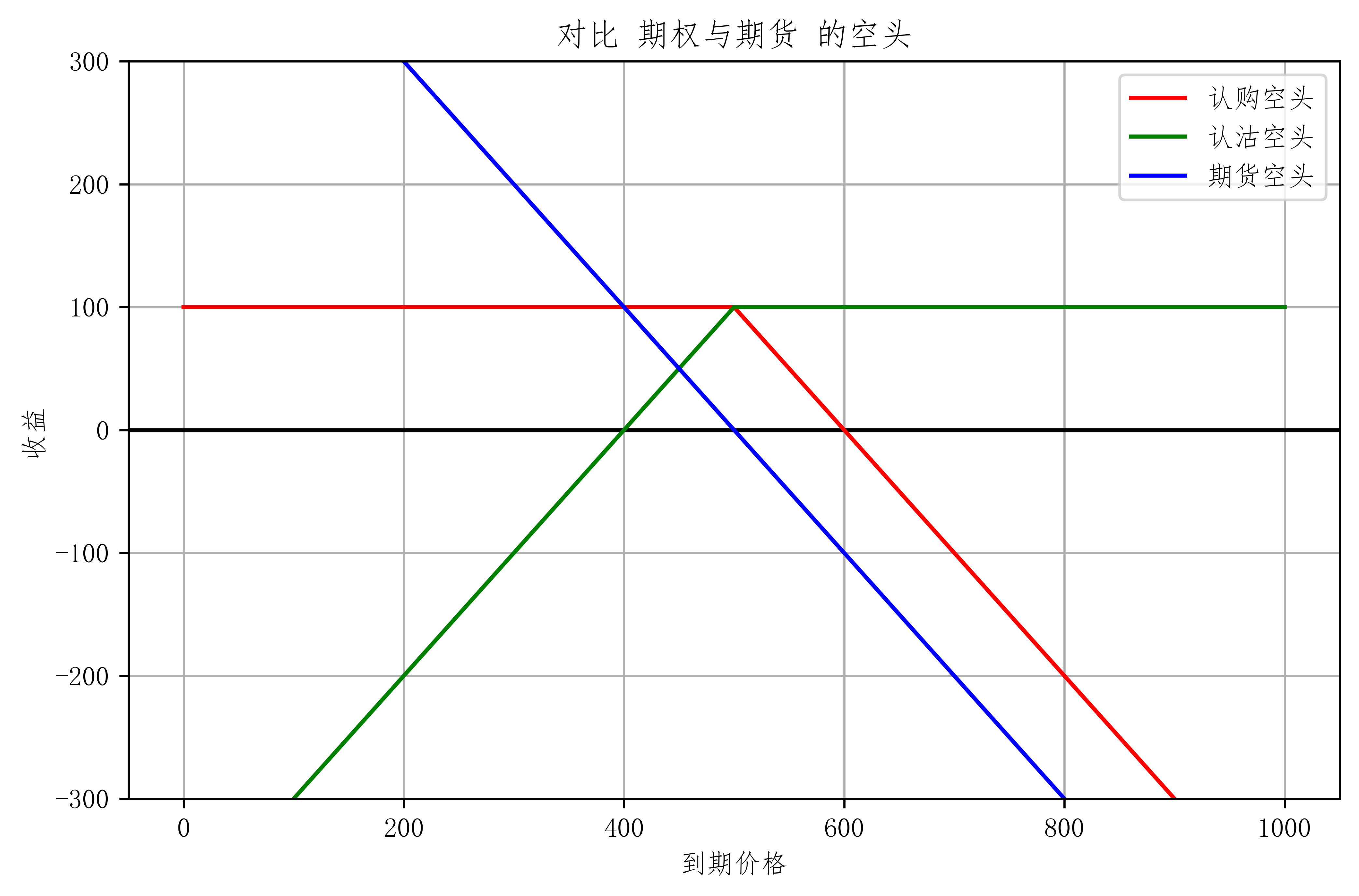• 在空头这张图 更可以直观看出，期货没有对波动有倾向性偏好。但做期权空头，说明 投资方 认为 波动趋近于平稳。

### 保证金

• 多头无需缴纳保证金
• 空头看涨保证金计算（两者取最大）：
1. $（结算价）期权费收入+标的资产收盘价值 \times 10 \% - 虚值额$
2. $（结算价）期权费收入+标的资产收盘价值 \times 5 \%$
• 空头看跌保证金计算（两者取最大）：
1. $（结算价）期权费收入+标的资产收盘价值 \times 10 \% - 虚值额$
2. $（结算价）期权费收入+行权价值 \times 5 \%$
• 例：
• 卖空一手当日结算价为 40点，行权价为 2200点的 一个月期 看涨期权，沪深300指数收盘价为 2150点： 最终缴纳的保证金为 20500元。
• 卖空一手当日结算价为 72点，行权价为 2200点的 一个月期 看跌期权，沪深300指数收盘价为 2150点： 最终缴纳的保证金为 28700元

### 欧式看涨期权价格曲线

• 运用 Black-Scholes 公式 绘制近似曲线
1. 看涨或看跌（c or p）
2. 标的资产现价（S0）
3. 期权执行价格（K）
4. 期权到期时间（t）
5. 适用的无风险利率（rf）
6. 适用的波动率（sigma）
7. 股利信息（本例中使用连续股利率dv）

#### 欧式期权价格曲线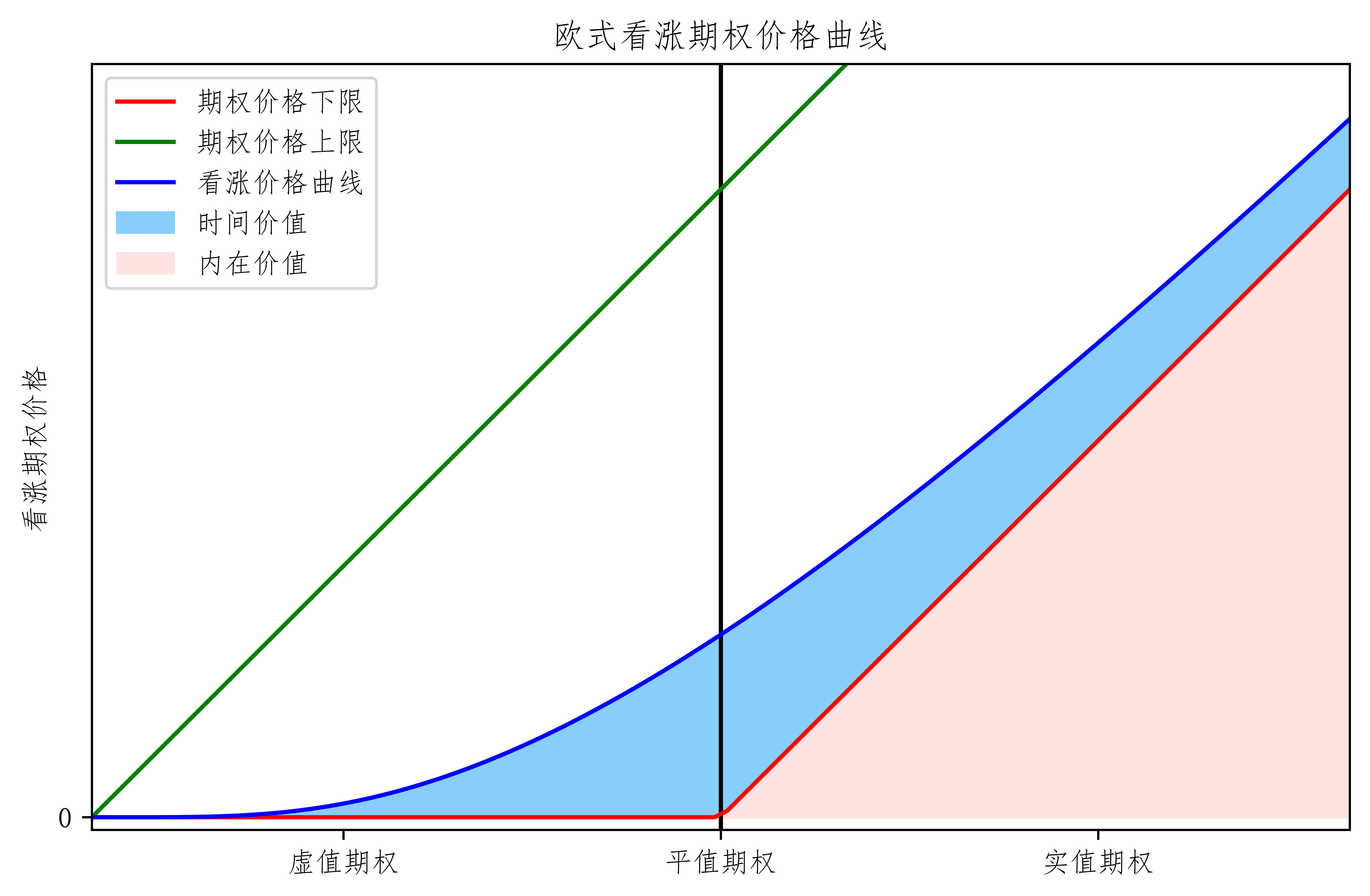#### 欧式期权看跌价格曲线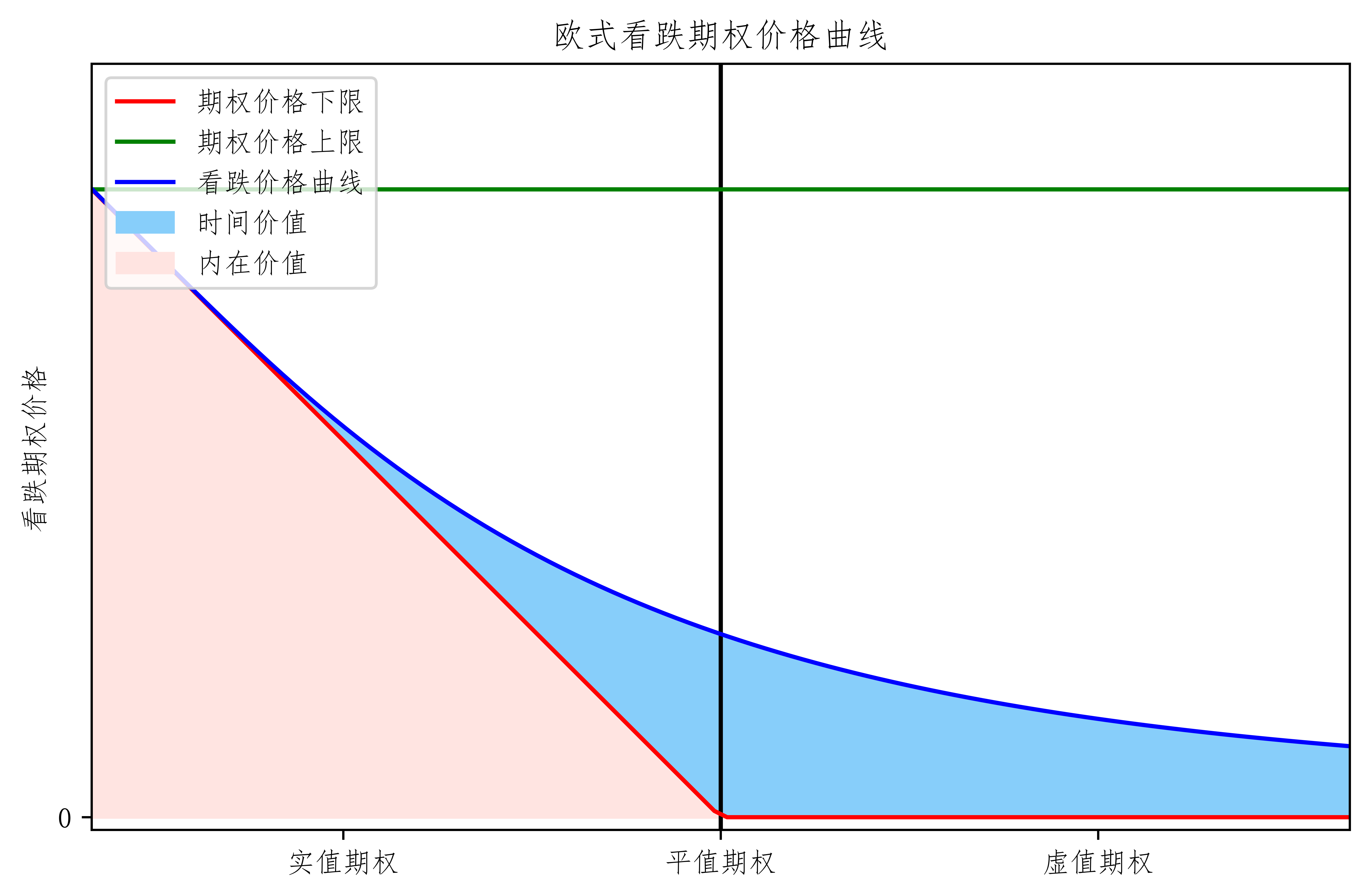• 从上面两图中可以看到，时间价值越接近 平值 它的价值越大。越往两边 它的价值自然减少。当然在其他因素不变的话，距离到期时间越短，它的时间价值就越低。呈抛物线加速衰减。

## 期权策略

### 单期权策略

• 单期权策略就是单买一种期权。

• 看空
• 预期大跌 <font color=blue>买</font>看<font color=green>跌</font>
• 预期不涨 <font color=purple>卖</font>看<font color=red>涨</font>
• 看多
• 预期大涨 <font color=blue>买</font>看<font color=red>涨</font>
• 预期不跌 <font color=purple>卖</font>看<font color=green>跌</font>上升下降

### 风险收益

#### 期权多头 风险收益

• 损失有限 收益理论无限 现实概率低

• 期权多头需要注意的是
• 最大亏损为 100%
• 波动率下降时可能导致亏损
• 天然存在 时间价值 time decay
• 行权价的选择

#### 期权空头 风险收益

• 损失理论无限 收益有限 现实概率高

• 在合理定价时 大概率盈利 小概率亏损
• 需要保证金约束
• 尽管如此 有小概率损失巨大

• 期权空头需要注意的是：
• 波动率上升可能导致亏损
• time decay 对空头有利
• 行权价的选择
• 认购多头合理定价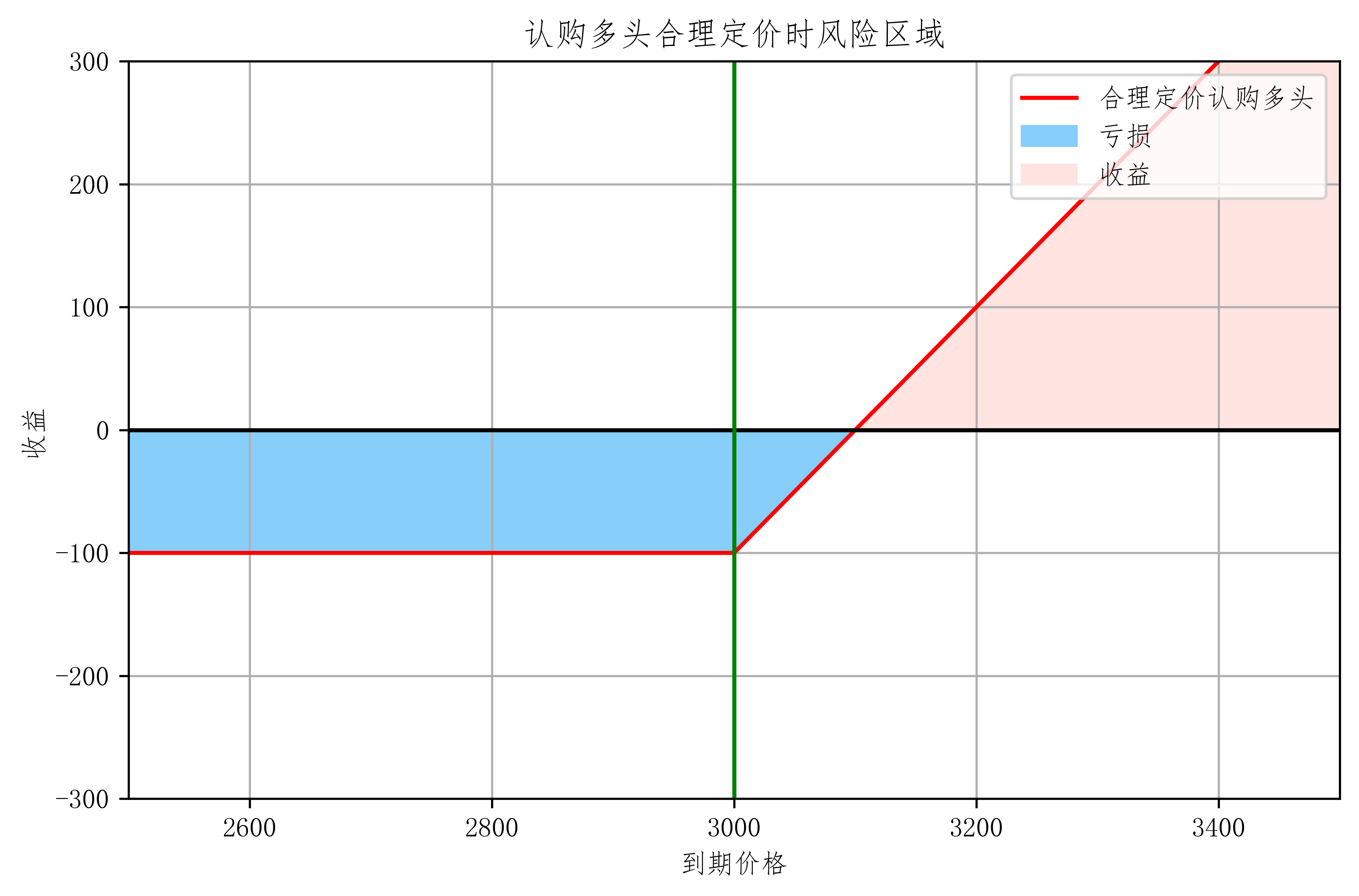• 认购多头期权高估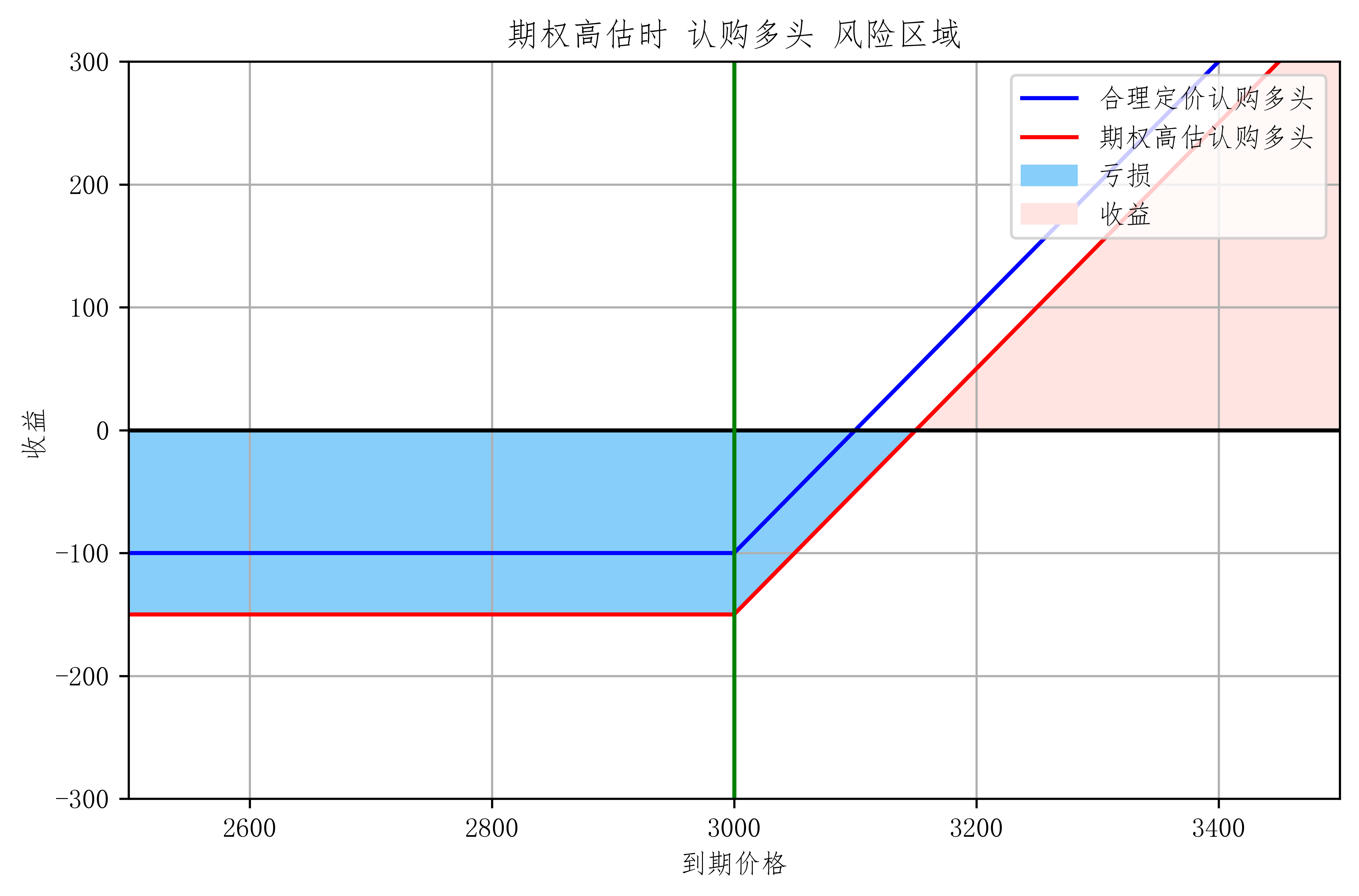• 认购多头虚值期权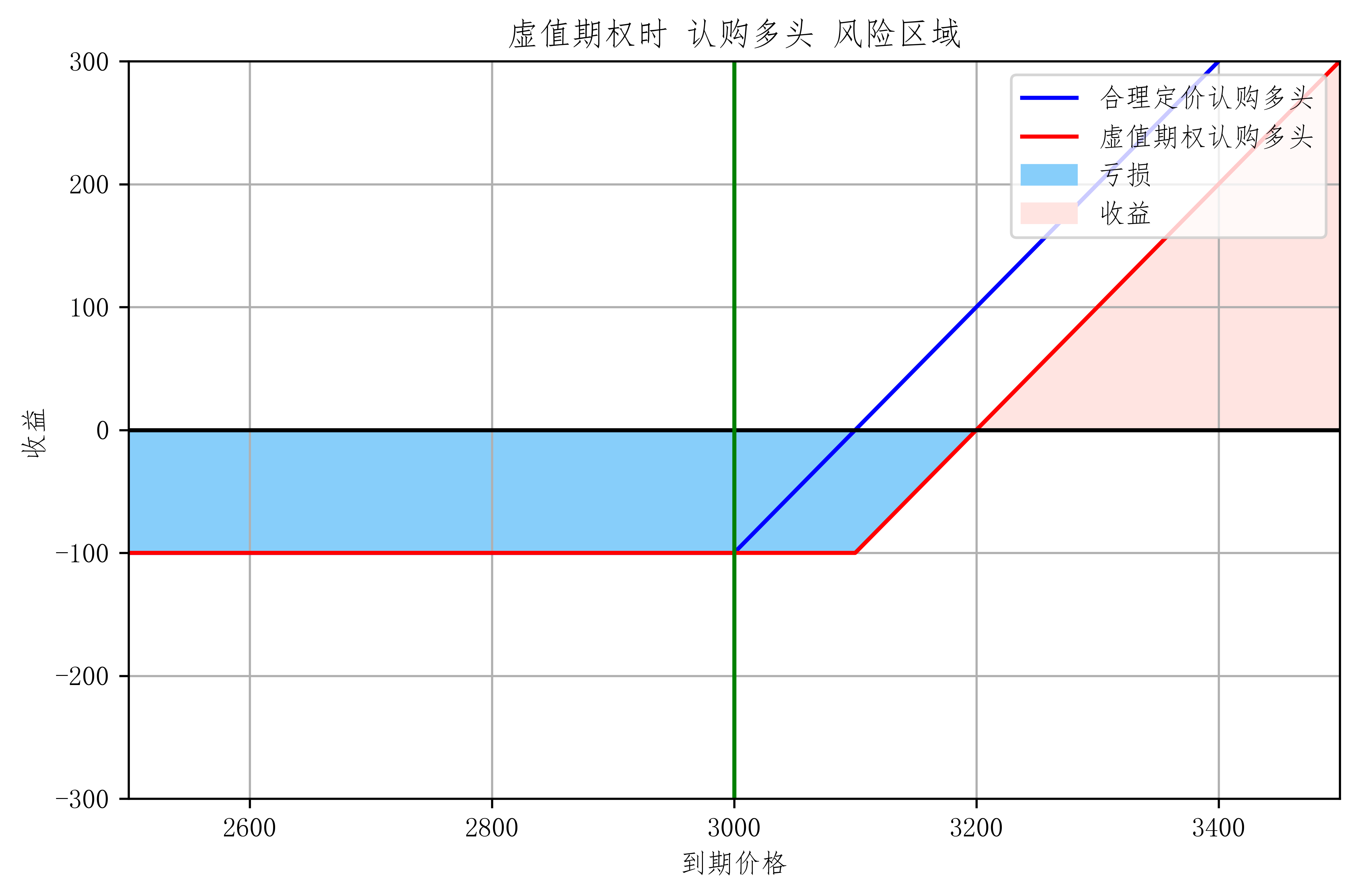### 跨式策略

#### 底部跨式

• 同时买入 看涨看跌期权

• 买入相同份额的 看涨看跌期权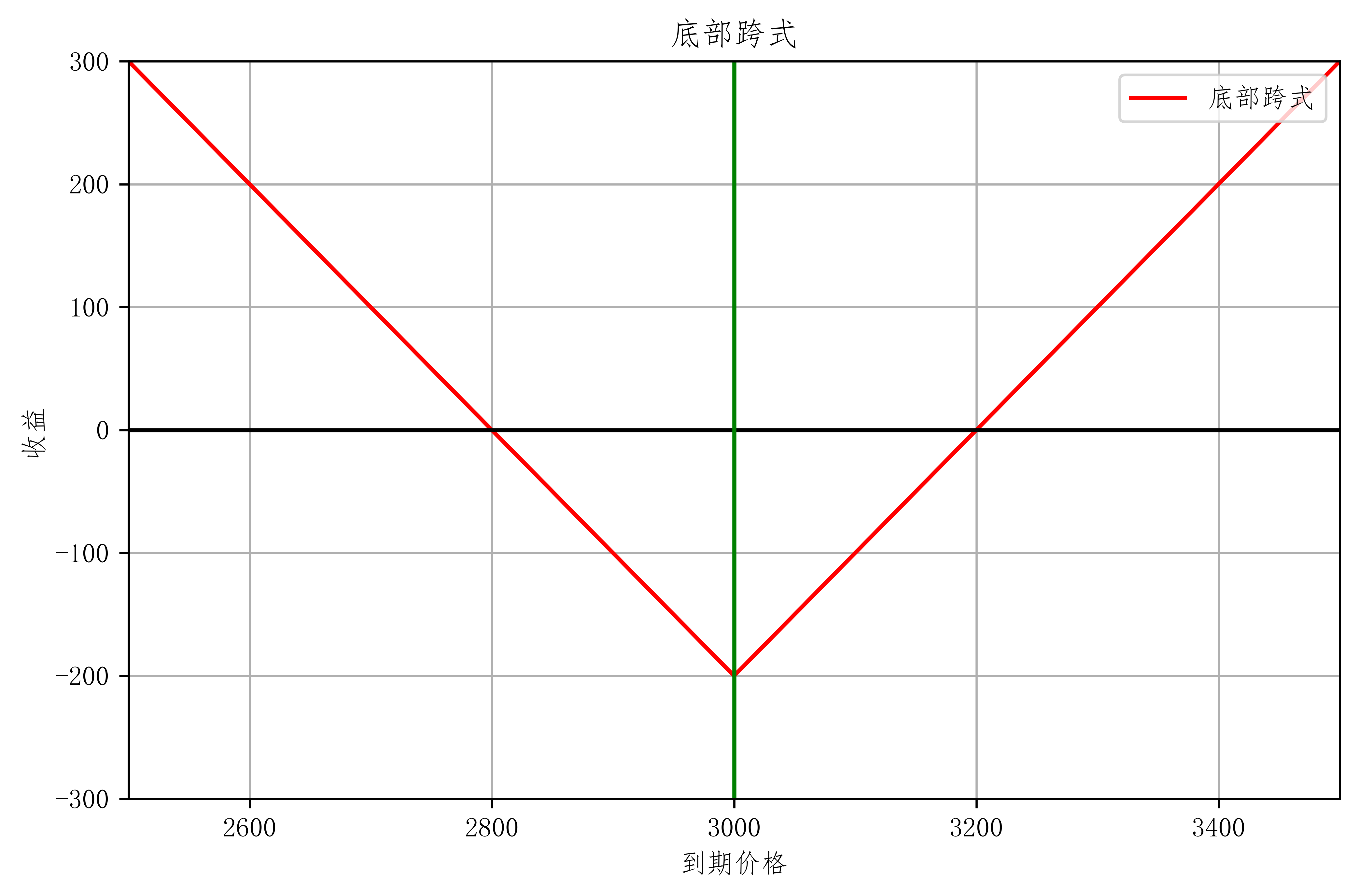• 亏损：期权费 $\times 2$
• 盈利：$[0,\infty)$
• 特征：
• 市场方向中性，波动大时获利
• 收益无限而风险有限
• 适用情形：
• 预期市场波动大
• 标的资产价格可能出现大的波动
• 预期有重大消息公布
• 暴跌或暴涨
• 策略变换：
• 顶部跨式策略
• 行权价
• 数量匹配
• 买入不同份额的 看涨看跌期权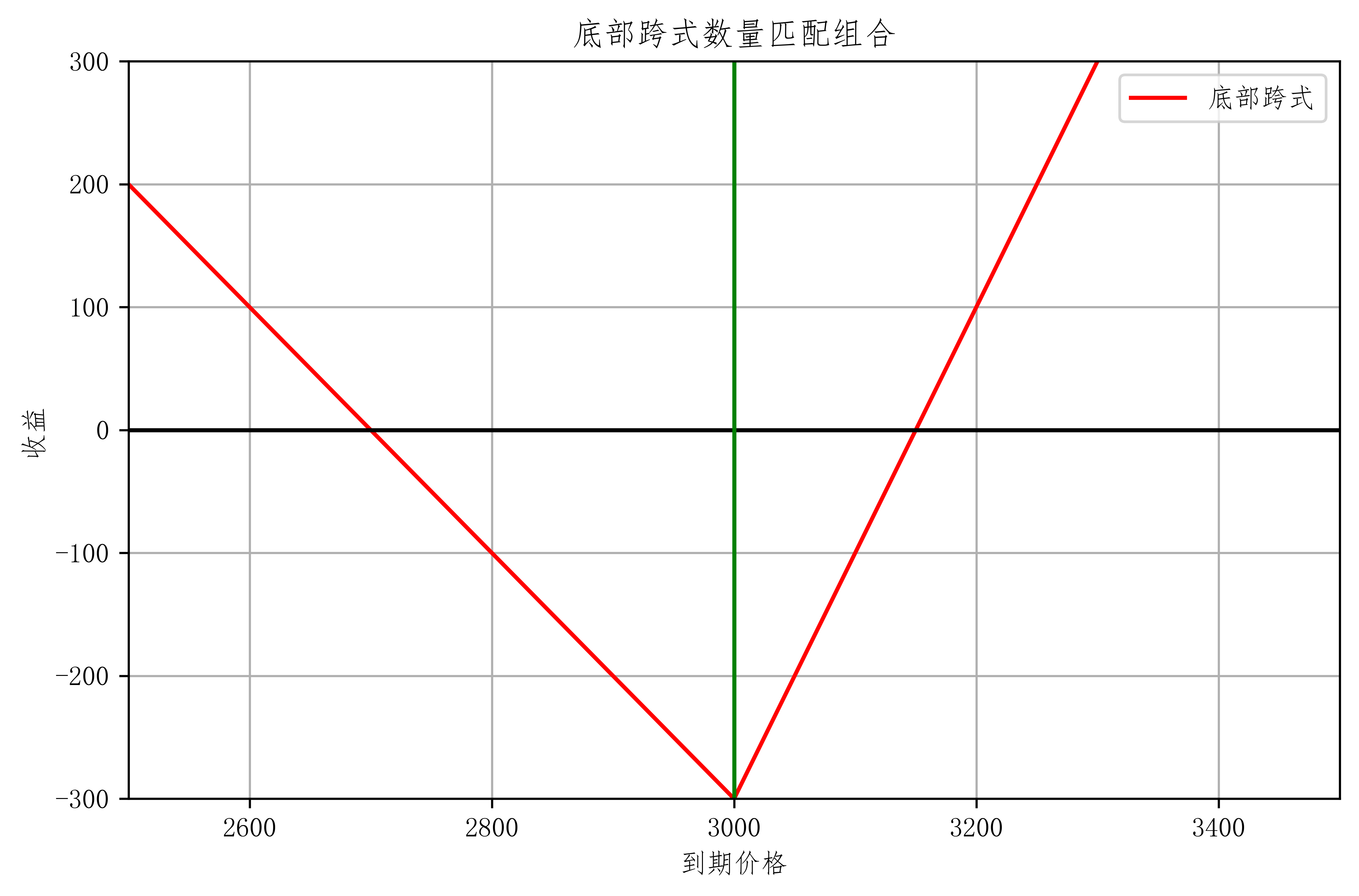#### 顶部跨式策略

• 同时卖出看涨看跌期权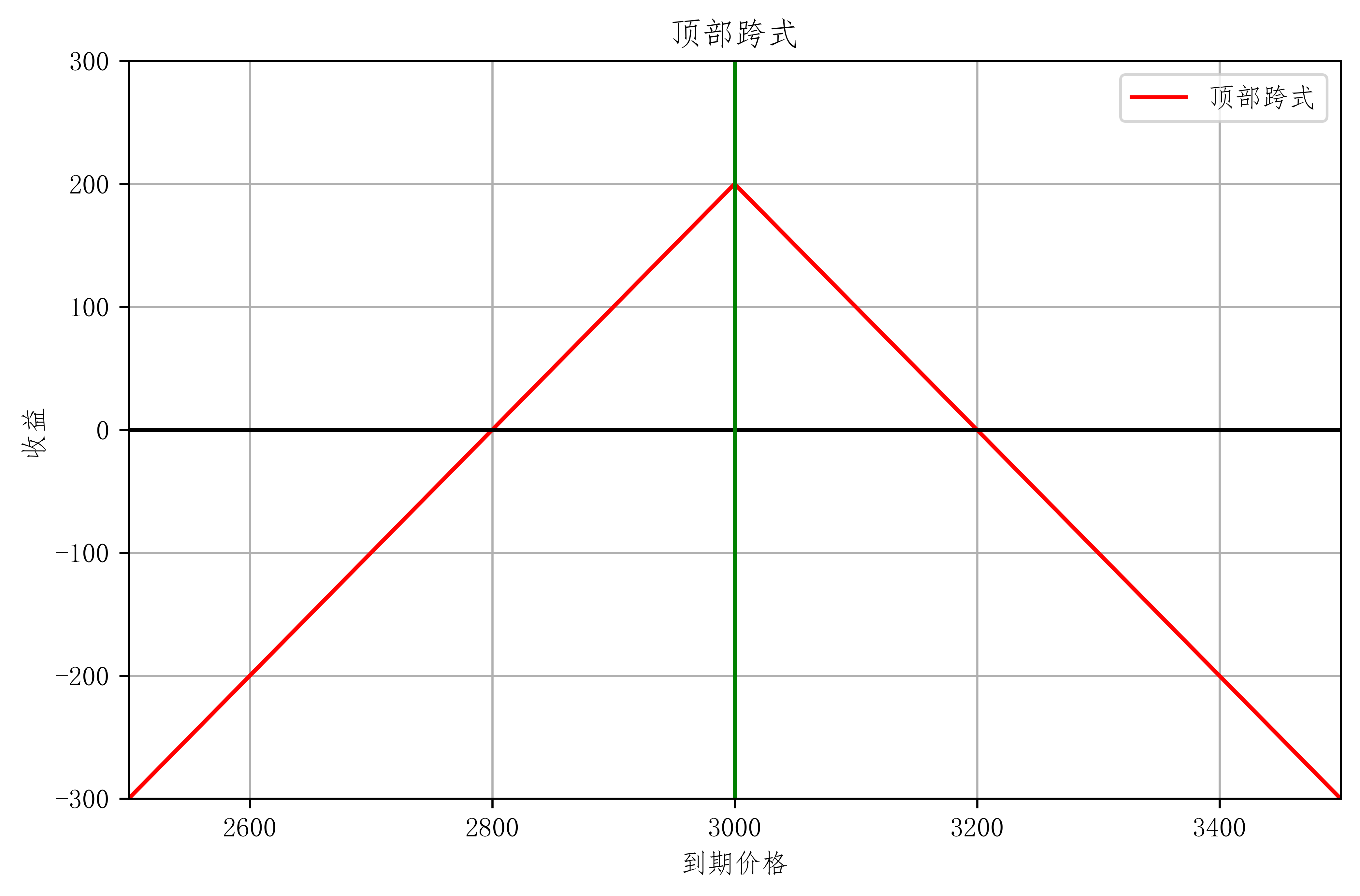### 勒式策略

• 因为跨式 行权价 成本偏高， 所以是对行权价偏高的一种调整
• 买入 两边 行权价 偏低的两个 看涨看跌期权。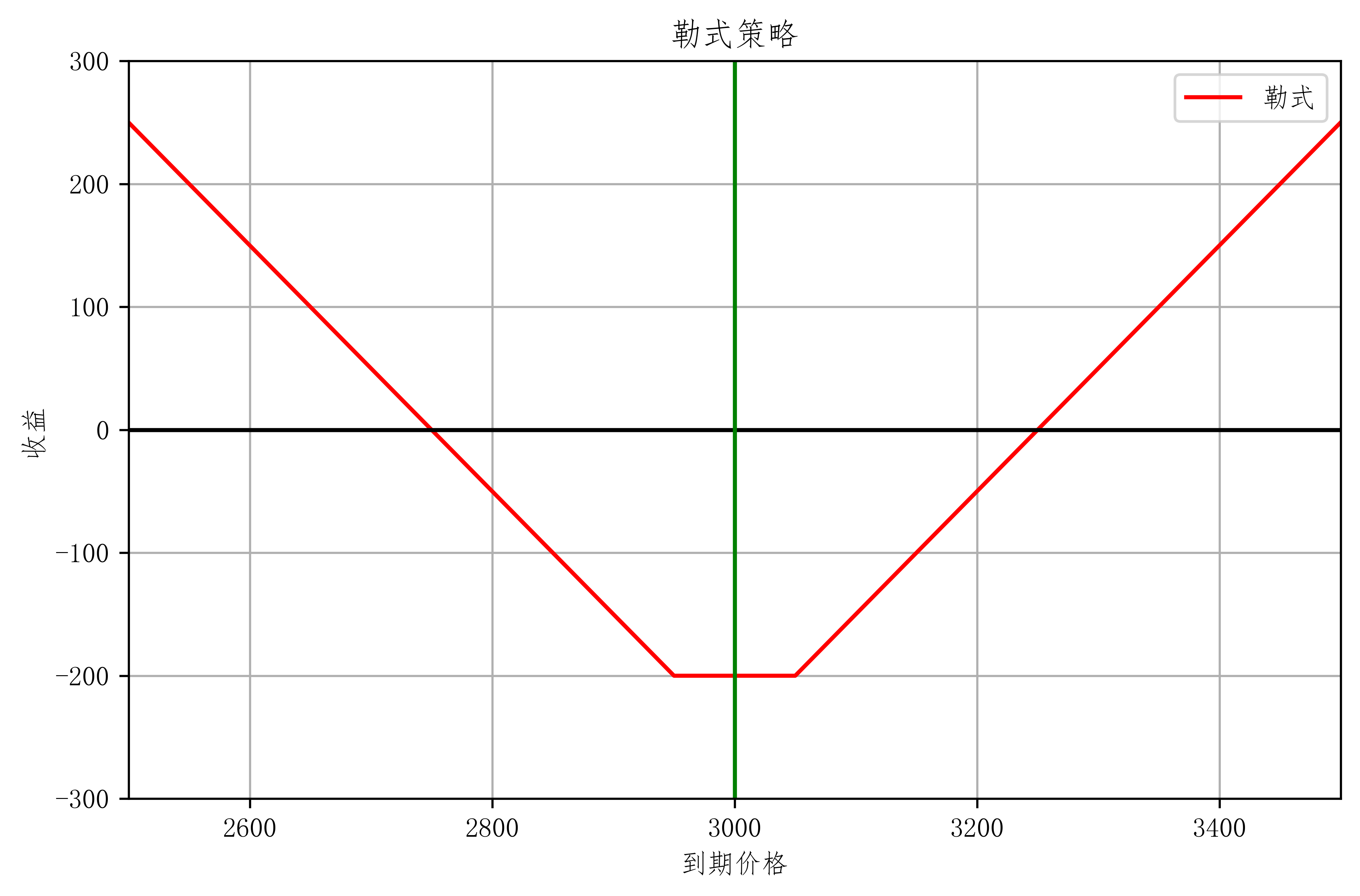• 亏损：期权费 $\times 2$
• 盈利：$[0,\infty)$
• 特征与适用情形：
• 与跨式相似
• 与跨式相比
• 初始成本下降，回报率上升
• 盈亏平衡点区间扩大

### 牛市价差组合

#### 看涨 牛市价差组合

• 卖出高价看涨 买入低价看涨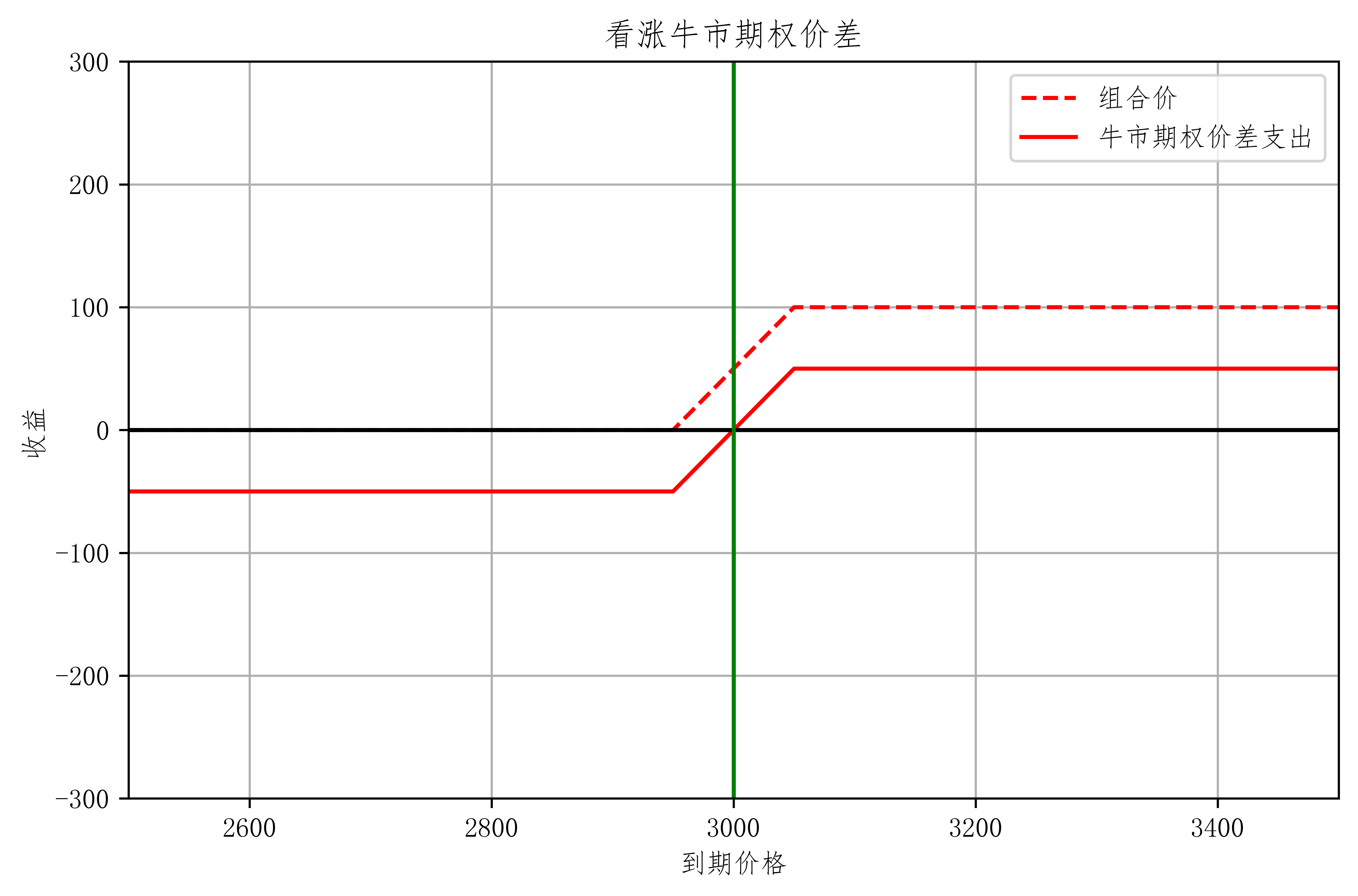• 策略特征
• 标的上涨时获利，下跌时亏损
• 风险收益均有限
• 与单买看涨期权相比
• 初期成本下降，最大亏损下降
• 温和上涨情况下：回报率相对较高
• 但收益空间有限
• 适用情形：预期标的资产价格温和上涨
• 当期权定价不合理时，会出现上图虚线的套利机会

#### 看跌牛市价差组合

• 买入低价看跌 卖出高价看跌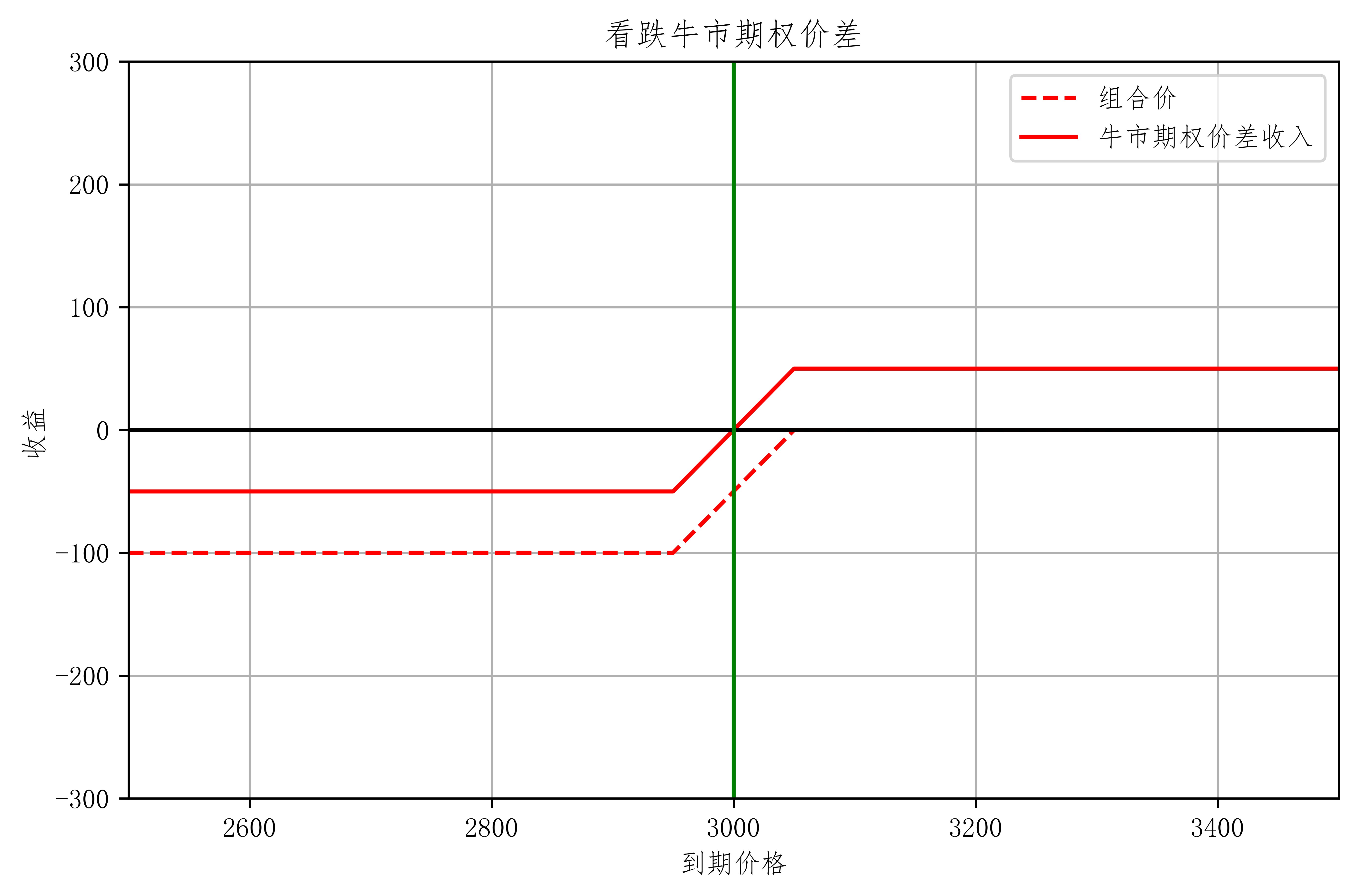• 适用情形：预期标的资产价格温和上涨
• 与看涨牛市价差相比
• 看涨：初期净支出，期末现金流回报 $\geq 0$
• 看跌：初期净收入，期末现金流回报 $\leq 0$
• 与单卖看跌相比
• 降低风险 也降低收入

### 熊市价差组合

#### 看跌熊市价差组合

• 买入高价看跌 卖出低价看跌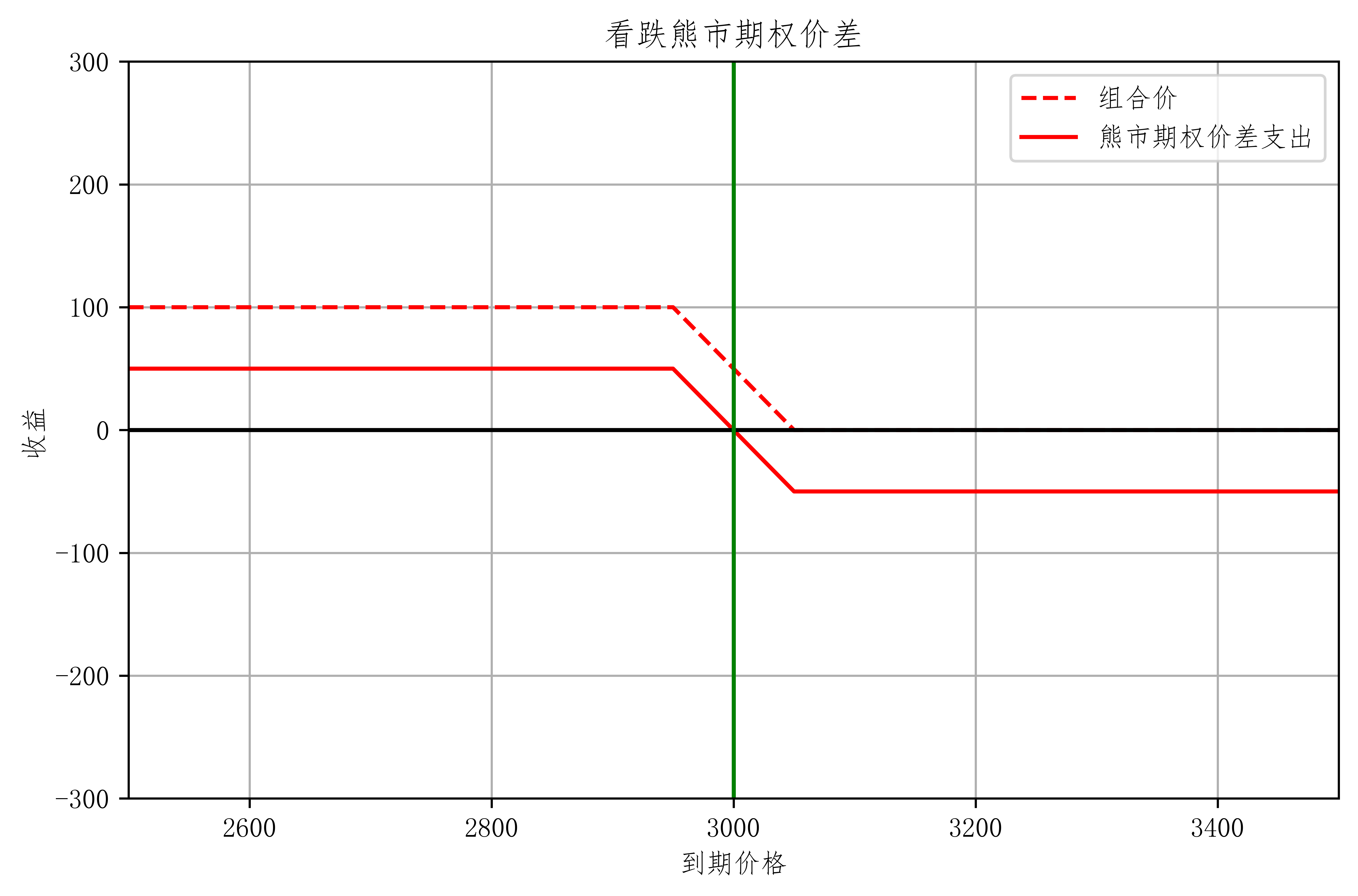• 策略特征
• 标的下跌时获利，上涨时亏损
• 风险收益均有限
• 与单买看跌期权相比
• 初期成本下降，最大亏损下降
• 温和下跌情况下：回报率相对较高
• 但收益空间有限
• 适用情形：预期标的资产价格温和下跌
• 当期权定价不合理时，会出现上图虚线的套利机会

#### 看涨熊市价差组合

• 买入高价看涨 卖出低价看涨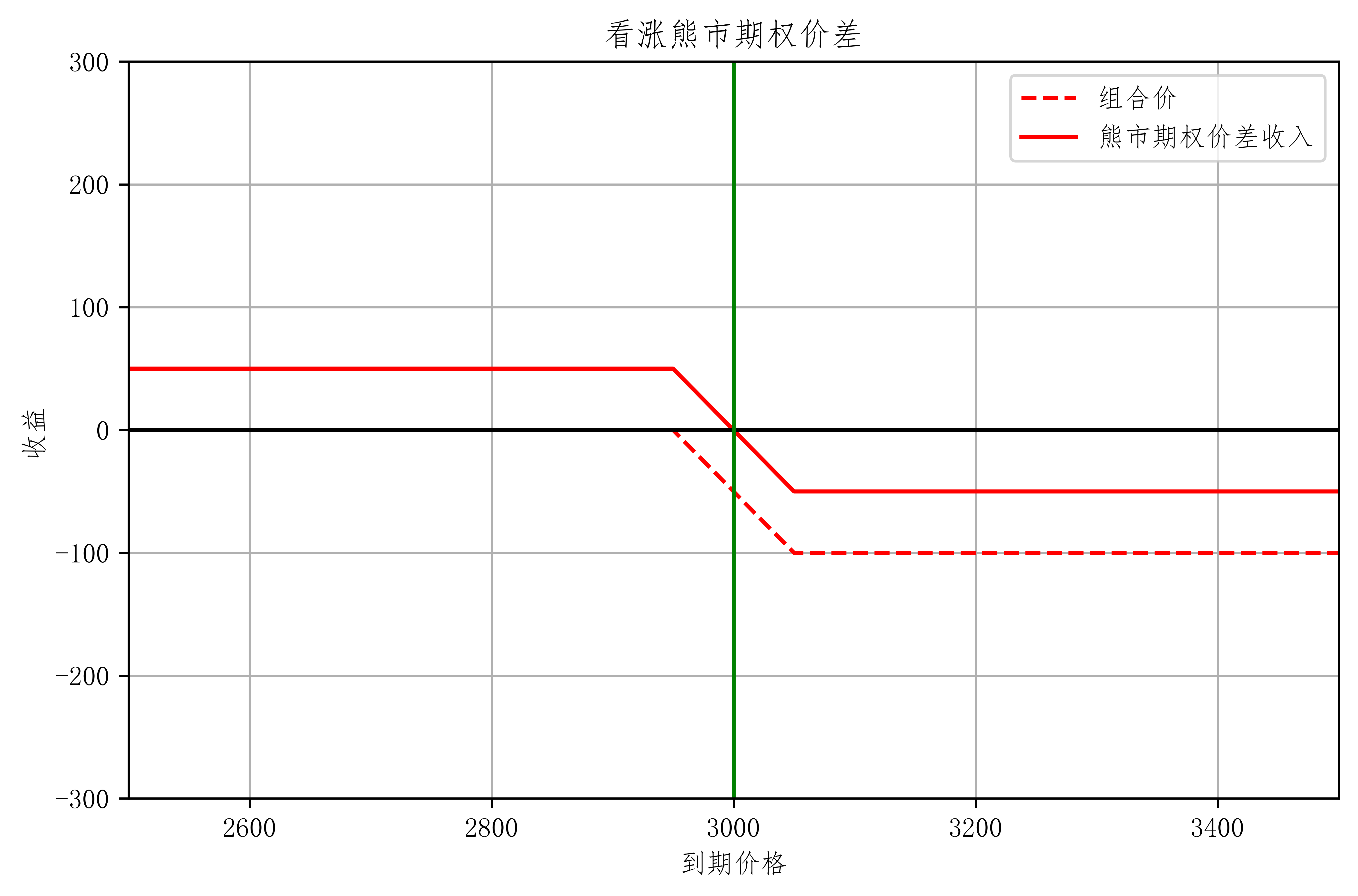• 适用情形：预期标的资产价格温和上涨
• 与看跌熊市价差相比
• 看跌：初期净支出，期末现金流回报 $\geq 0$
• 看涨：初期净收入，期末现金流回报 $\leq 0$
• 与单卖看涨相比
• 降低风险 也降低收入

### 蝶式组合

#### 正向蝶式价差

• 买对称高低行权价，卖出双份中行权价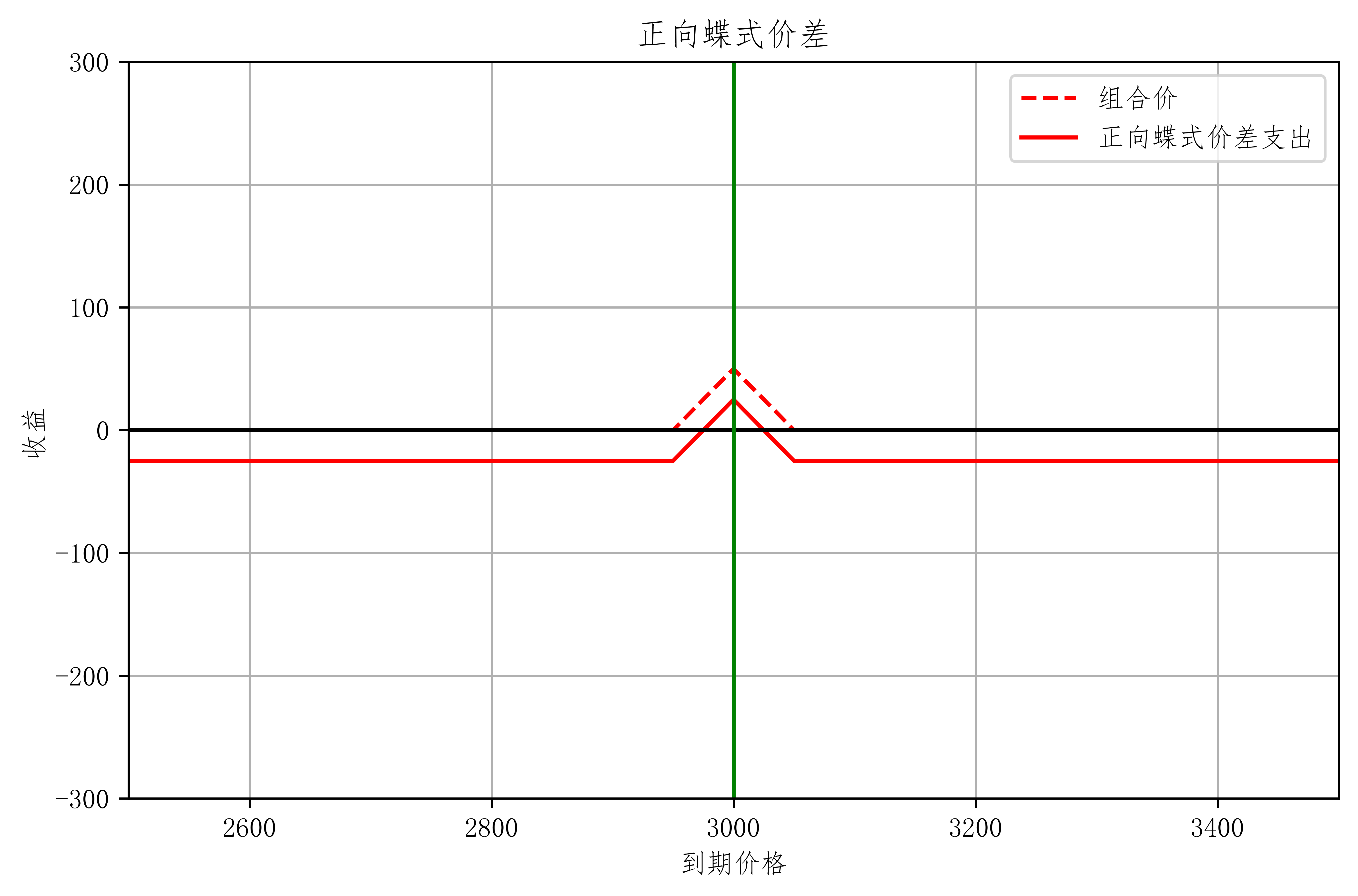• 策略特征
• 市场低波动时高回报率
• 风险收益均有限
• 适用情形：预期市场方向中性、低波动率
• 策略变化
• 看跌期权构造 理论上应相同
• 反向蝶式
• 行权价
• 套利

#### 反向蝶式策略

• 卖对称高低行权价，买双份中行权价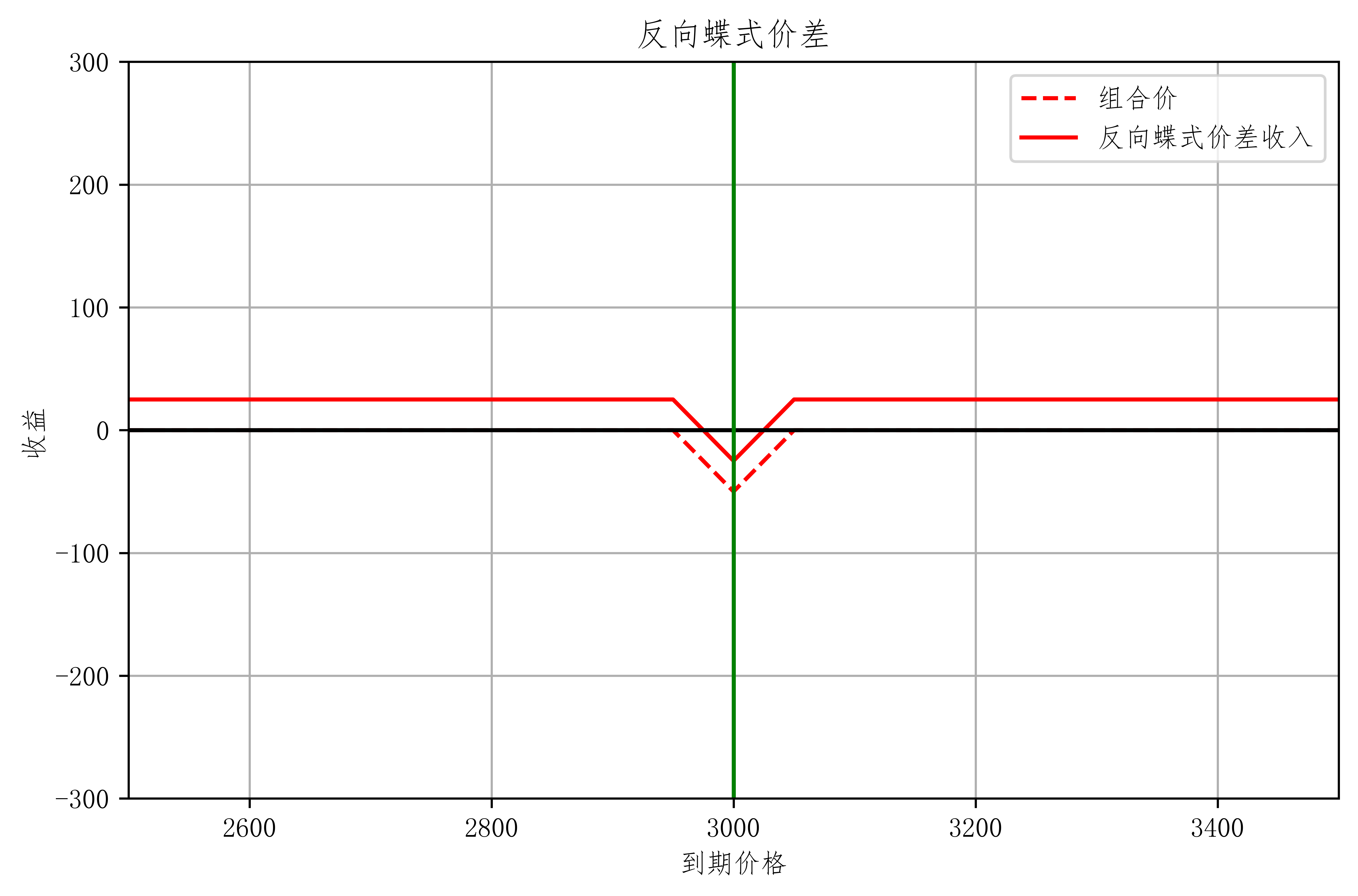• 策略特征
• 市场高波动时高回报率
• 风险收益均有限
• 适用情形：预期市场高波动率

## PCP

### PCP 平价原理

• Put-Call Parity（PCP）
• 在欧式期权 标的资产不付红利的情况下

c 看涨期权
p 看跌期权
S 标的资产现价
X 现金
r 无风险利率
T 到期时刻
t 当前时刻

### PCP 现货

• 组合
1. 看涨期权 $c$ + 现金（行权价的无风险贴现值 $\frac{X}{1+r(T-t)}$）
2. 看跌期权 $p$ + 标的资产 $S$
• 当到期时刻 T 时：
• 当$S_T > X$: 组合1价值 $S_T$ 组合2价值 $S_T$
• 当$S_T \leq X$: 组合1价值 $X$ 组合2价值 $X$
• 组合1 == 组合2
• 在无套利的情况下 当前t时刻的两个组合价值也应该相等
• $c + \frac{X}{1+r(T-t)} = p + S$
• 组合1 == 组合2

#### 正向套利操作 （Forward）

• 卖出看涨期权($+c$)，买入看跌期权和股票($-p$)，借入所需资金($-S_0$)，最终需还 $(p + S_T-c)$ 终值
• 到期时，无论股指高于或低于行权价 $X$ ，投资者均以 $X$ 元卖出手中股票，获得 $X-S_T$，与手中股票多头抵消，最终获得 $X$
• 获利: $X-(p+S_T-c)$
• 若扣除 税费等其他因素 仍然赚钱 那么 这交易策略现实可行

#### 反向套利操作 （Reversal）

• 买入看涨期权($-c$)，卖出看跌期权和股票($+p$)，贷出资金($+S_0$)，最终收入 $(p + S_0-c)$ 终值
• 到期时，无论股指高于或低于行权价 $X$ ，投资者均以 $X$ 元买入期权股票，获得 $S_T-X$，与手中股票空头抵消，最终结果 $-X$
• 获利: $(p+S_T-c)-X$
• 若扣除 税费等其他因素 仍然赚钱 那么 这交易策略现实可行

### PCP 期货套利

F 期货价格

• PCP 平价组合
• 期货 交易成本较低 流动性较好

## 策略构造

### 保护性看跌策略

• 情形：假如投资者 买入了一份股票 又担心它会下跌
• 股票多头 + 虚值或平价看跌期权多头
• 用 PCP 平价解释：
• 买入股票 $S + p$ 买看跌期权 = 看涨多头 $c$
• 所以构造出了看涨多头股票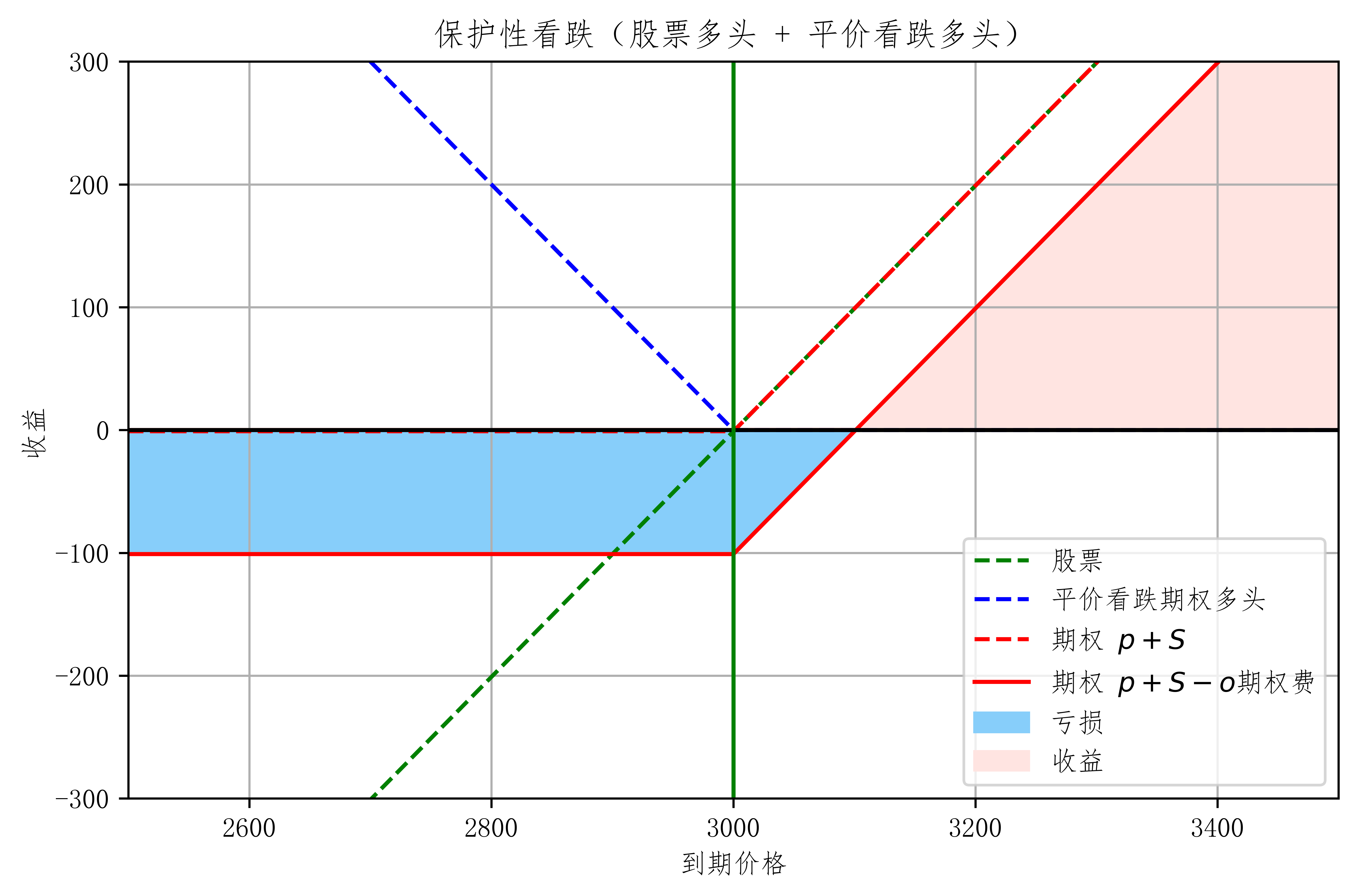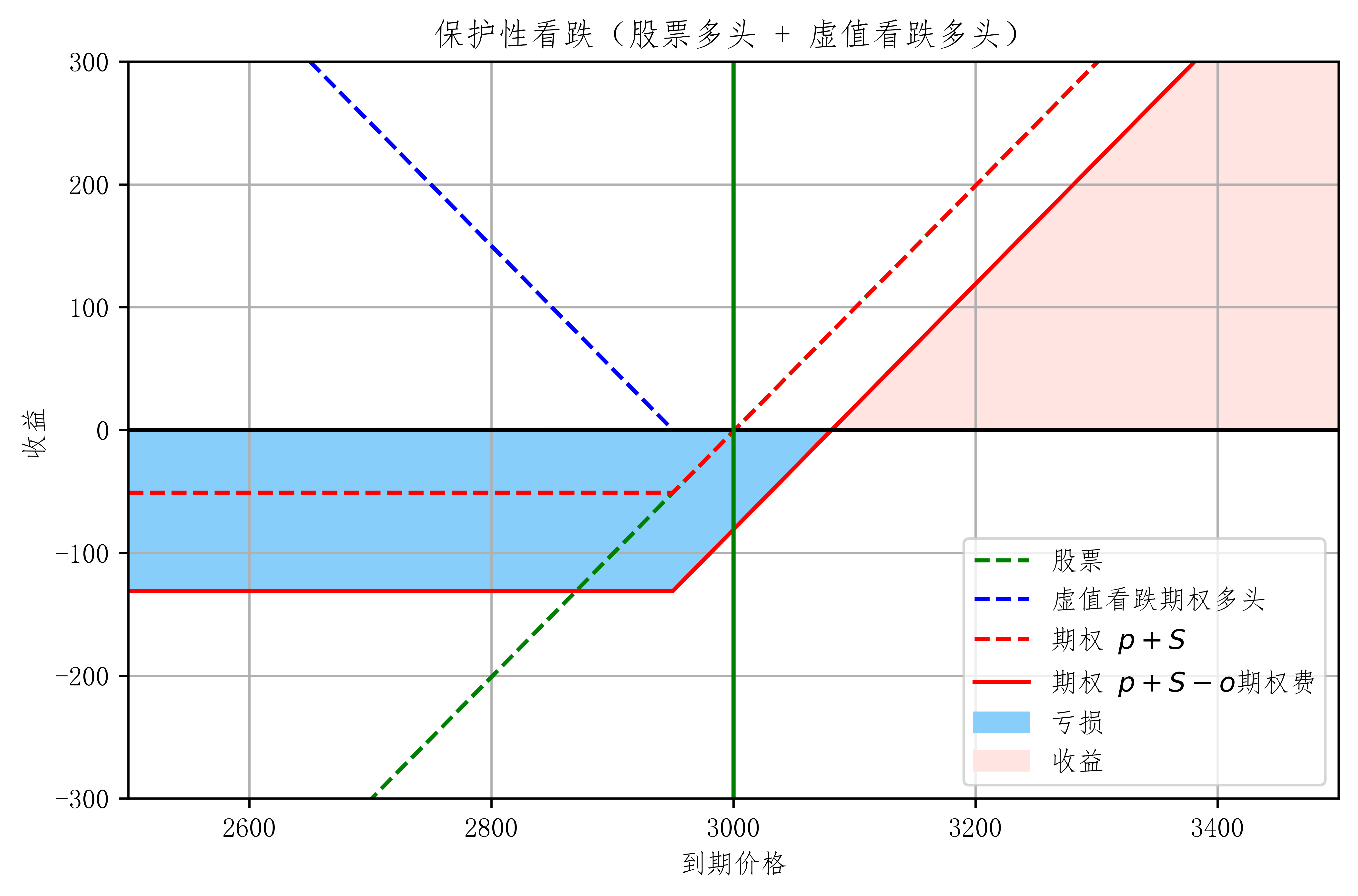• 策略特征
• 收益无限 风险有限
• 相对仅持有现货
• 保留上升空间，保护下跌风险
• 支付成本：盈亏平衡点提高，上涨时相对盈利少
• 适用情形：保守牛市预期
• 用看跌期权多头保护股票价格下跌风险

### 备兑看涨策略

• 持有现货 卖出平价或虚值看涨期权

• 股票 + 平价看涨期权空头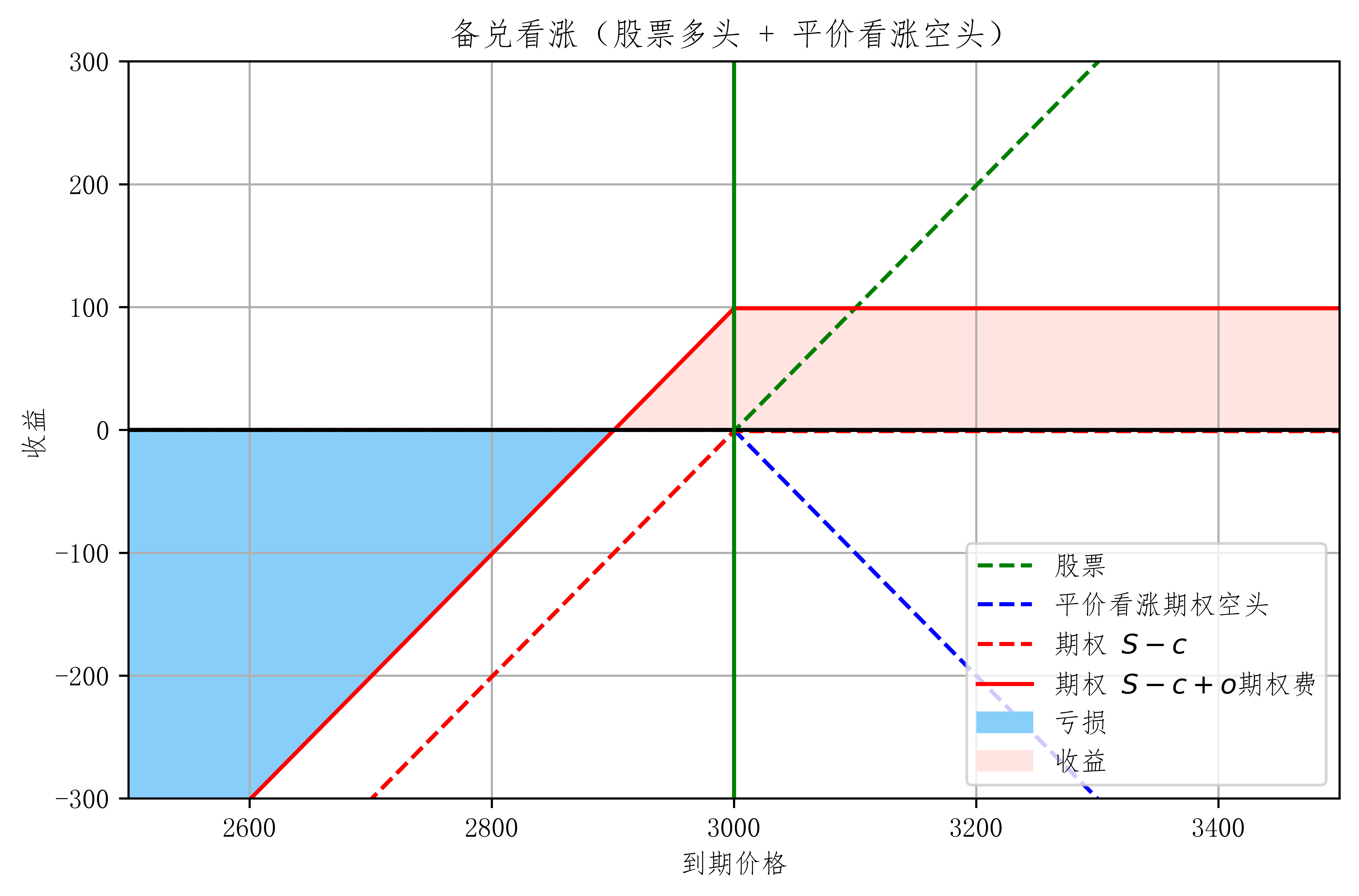• 股票 + 虚值看涨期权空头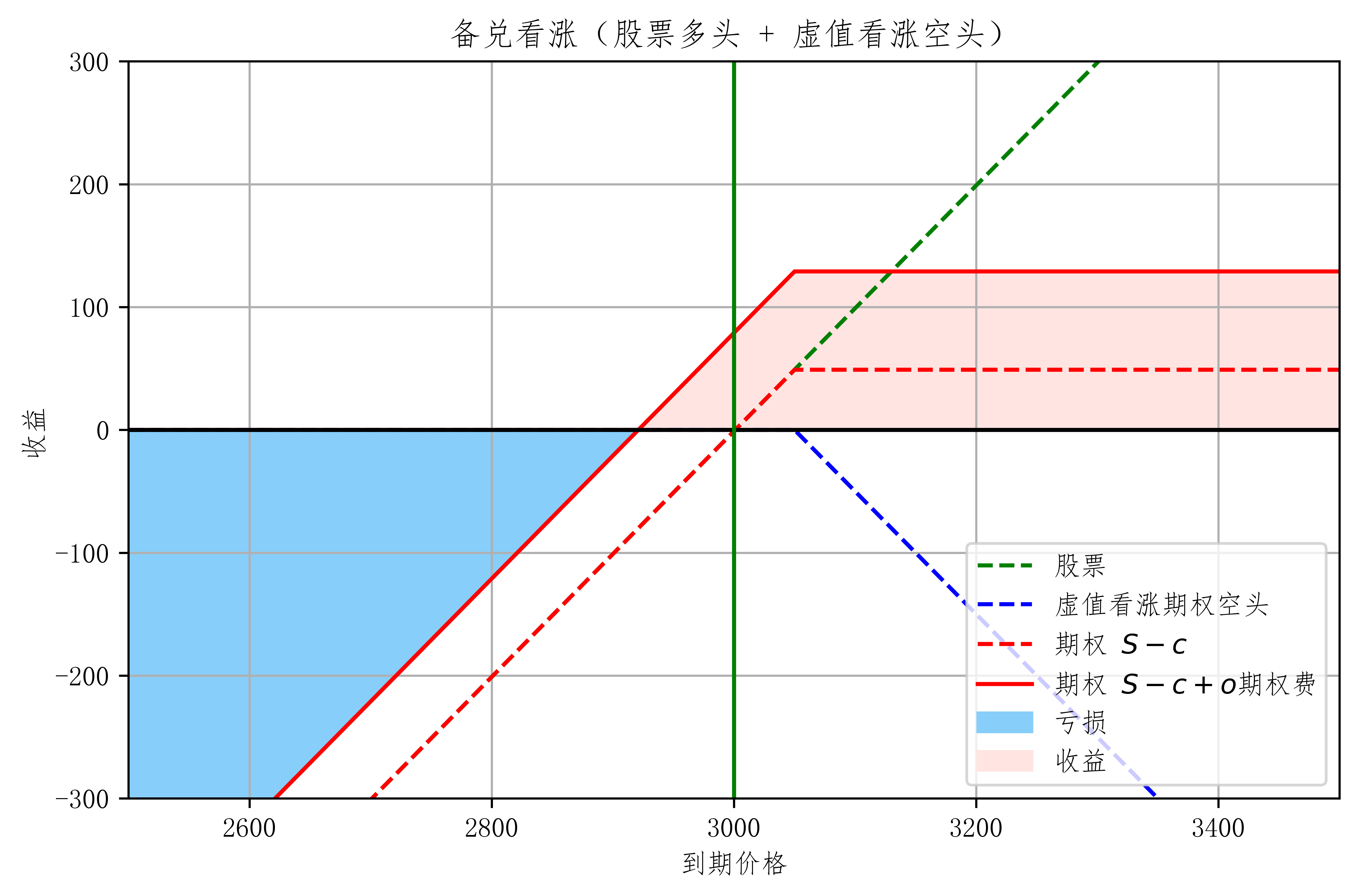• 策略特征
• 收益有限而风险较大
• 相对仅有现货
• 下跌风险降低：增加收入；盈亏平衡点下降
• 上升空间受限
• 适用情形
• 增加收入（因各种原因无法出售）
• 约束盈利离场价位

### 期权风险分析

• 期权价格同时受到 标的价格方向 和 波动率 的影响
• 期权空头：
• 最大收益为期权费收入：大概率
• 最大亏损无限或较大：小概率
• 但小概率事件一旦发生，可能亏损严重
• 保证金制度的约束（保证金不足需要追加）
• 期权多头：
• 盈利时汇报率较高，且最大亏损为期权费收入
• 亏损是大概率事件，且可能亏损 100%
• 无保证金制度约束
• 谨防虚值期权爆炒风险：期权与权证不同

### Black-Scholes-Merton 公式

• 欧式定价基准模型
• 无红利欧式期权定价公式为例：

c 看涨期权价格
p 看跌期权价格
S 标的资产现价
X 行权价
r 无风险利率
T 到期时刻
t 当前时刻
𝜎 波动率
N()为标准正态分布累积分布函数

• 用 Python 把这公式写出来：
import numpy as np
from scipy.stats import norm

class BlackScholes:
def __init__(self, S0, X, r, T, sigma=0.3,t=0):
self.S0 = S0
self.X = X
self.r = r
self.sigma = sigma
self.dT = T-t

def d1(self):
return(np.log(self.S0/self.X)+(self.r+self.sigma**2/2)*(self.dT))/(self.sigma*np.sqrt(self.dT))

def d2(self):
return self.d1()-self.sigma*np.sqrt(self.dT)

def calc(self, call_put):
if call_put in {'c','C','call','Call','CALL'}:
return self.S0 * norm.cdf(self.d1())- \
self.X*np.exp(-self.r*self.dT)*norm.cdf(self.d2())
elif call_put in {'p','P','put','Put','PUT'}:
return self.X*np.exp(-self.r*self.dT)*norm.cdf(-self.d2())- \
self.S0 * norm.cdf(-self.d1())
raise NameError('Must be call or Put!',call_put)

def imp_vol(self,call_put,mktprice):
price = 0
sigma = 0.3
up, low = 1,0
loop = 0
while abs(price-mktprice)>1e-6 and loop<50:
price = BlackScholes(self.S0,self.X,self.r,self.dT,sigma).calc(call_put)
if (price-mktprice)>0:
up = sigma
sigma = (sigma+low)/2
else:
low = sigma
sigma = (sigma+up)/2
loop+=1
return sigma

• 和市场定价不符合因为它假设
• 市场可复制和无套利
• 可卖空、无税费、连续交易、证券可分
• 无套利
• 标的资产服从连续的几何布朗运动
• 标的无跳动
• 波动率为常数
• 无风险利率为常数

• 计算隐含的波动率
• 估计希腊字母（偏导）
• 定价基准

## 隐含波动率

### 波动率 $\sigma$

• 波动率 $\sigma$
• 波动率为期权价格影响的一个重要因素
• 没有波动，期权就没有存在的价值
• 不可观测变量
• 在统计中的对应概念：价格（对数）收益率的年化标准差

$N$ 天数
$R_n$ 第n天的收益率
$\bar{R}$ 平均收益率
$242$ 一年中国交易天数

### 波动率分类

• 历史波动率 Historical volatility & 未来波动率 Future Volatility

• 历史波动率法：
• 基于标的资产已发生的历史价格数据估计波动率：
• 标准差
• 极差波动率
• 已实现波动率 Realized Volatility (RV)
• 根据历史波动率预测未来的波动率：
• 预测值 = 历史值
• GARCH, EWMA
• HAR-RV
• 隐含波动率法：
• 从期权价格倒推市场预测未来波动率
• Black-Scholes 隐含波动率
• 无模型隐含波动率 (如 VIX)

### 隐含波动率

• Implied Volatility

• 隐含波动率偏高 $\to$ 期权价格偏高
• 隐含波动率偏低 $\to$ 期权价格偏低

### 实例

• 来看一下 具体实例：
1. 导入 需要的库（还是实用 tushare 的数据）

2. 以上证 50 ETF 为例

• 获取上证 50 ETF 数据
opt_name = pro.opt_basic(exchange='SSE', fields='ts_code,name,exercise_type,list_date,delist_date')

ts_code name exercise_type list_date delist_date
0 10000579.SH 华夏上证50ETF期权1604认购2.15 欧式 20160225 20160427
1 10000108.SH 华夏上证50ETF期权1505认购2.65 欧式 20150326 20150527
2 10000111.SH 华夏上证50ETF期权1505认沽2.55 欧式 20150326 20150527
3 10001067.SH 华夏上证50ETF期权1712认购3.24 欧式 20171123 20171227
4 10001068.SH 华夏上证50ETF期权1712认沽3.24 欧式 20171123 20171227
1. 提取 需要的期权名 到期日期 价格 认购标签等
• 当然 Tushare 本身带有 这一系列简单的提取方式，在上式 修改 fields 所需参数 即可。
# 把 name 里的数据提取出来
opt_name['new_name']= opt_name['name'].str.extract(r'([\u4e00-\u9fa5]+)') # 提取期权名
opt_name['delist'] = opt_name['name'].str.extract(r'(期权)(\d+)').astype(int) # 期权到期日期
opt_name['type']= opt_name['name'].str.extract(r'(\d+)') # 提取期权类型 300 或 50
opt_name['callput']= opt_name['name'].str.extract(r'(\w\w)(\d+\.\d+)')# 认购或认沽
opt_name['price'] = opt_name['name'].str.extract(r'(\d+\.\d+)').astype(float) # 价格
opt_name.drop(columns=['name'],inplace=True)
opt_name['callput'].replace({'认购':'Call', '认沽':'Put'},inplace=True)

ts_code exercise_type list_date delist_date new_name delist type callput price
0 10000579.SH 欧式 20160225 20160427 华夏上证 1604 50 Call 2.15
1 10000108.SH 欧式 20150326 20150527 华夏上证 1505 50 Call 2.65
2 10000111.SH 欧式 20150326 20150527 华夏上证 1505 50 Put 2.55
3 10001067.SH 欧式 20171123 20171227 华夏上证 1712 50 Call 3.24
4 10001068.SH 欧式 20171123 20171227 华夏上证 1712 50 Put 3.24
1. 以 昨天 2020年 4 月 29 日数据为例
• 提取 2020年 4 月 29 日期权交易数据
# 找到 4月 29日的期权交易数据
DATE = '20200429'

1. 合并交易名与交易具体数据
new_date = pd.merge(opt_name,opt_date,on=['ts_code']) # 正在交易的名字和4月29日的数据交集

1. 提取 上证2020年06月到期的 50ETF 认购 4月29日 数据
• 并按行权价 排序
call_date_2006 = new_date.query('delist == 2006 and type == "50" and callput == "Call"').sort_values(by='price')

ts_code exercise_type list_date delist_date new_name delist type callput price trade_date ... pre_settle pre_close open high low close settle vol amount oi
20 10002421.SH 欧式 20200320 20200624 华夏上证 2006 50 Call 2.35 20200429 ... 0.46 0.4327 0.4300 0.4542 0.4300 0.4498 0.479 0.0221 985895.0 2353.0
46 10002401.SH 欧式 20200319 20200624 华夏上证 2006 50 Call 2.40 20200429 ... 0.41 0.3842 0.3816 0.4050 0.3808 0.3950 0.429 0.0043 170508.0 2079.0
47 10002402.SH 欧式 20200319 20200624 华夏上证 2006 50 Call 2.45 20200429 ... 0.36 0.3373 0.3362 0.3576 0.3362 0.3549 0.379 0.0104 364896.0 1075.0
96 10002291.SH 欧式 20200204 20200624 华夏上证 2006 50 Call 2.50 20200429 ... 0.31 0.2909 0.2889 0.3130 0.2864 0.3069 0.329 0.0162 493784.0 2725.0
97 10002292.SH 欧式 20200204 20200624 华夏上证 2006 50 Call 2.55 20200429 ... 0.26 0.2458 0.2430 0.2673 0.2430 0.2620 0.279 0.0127 328143.0 2058.0

5 rows × 21 columns

1. 为了解释 标的资产现价 与 行权价的关系，绘制图表
• 当天股票收盘价为 2.829 元
• 可见 越接近 标的资产现价 它的时间价值或者说 期权价 越高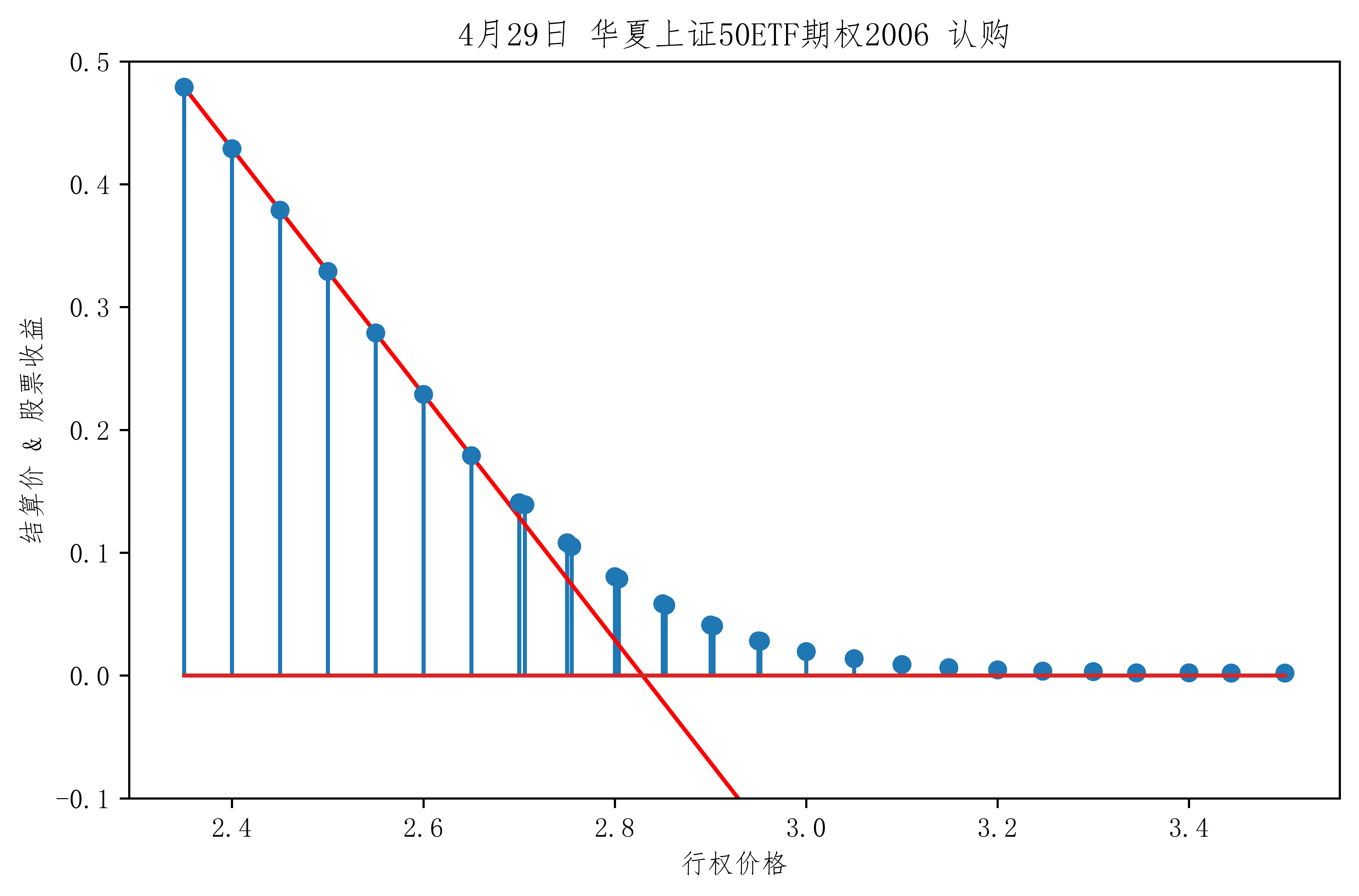1. 具体行权价与期权价到期收益情况绘图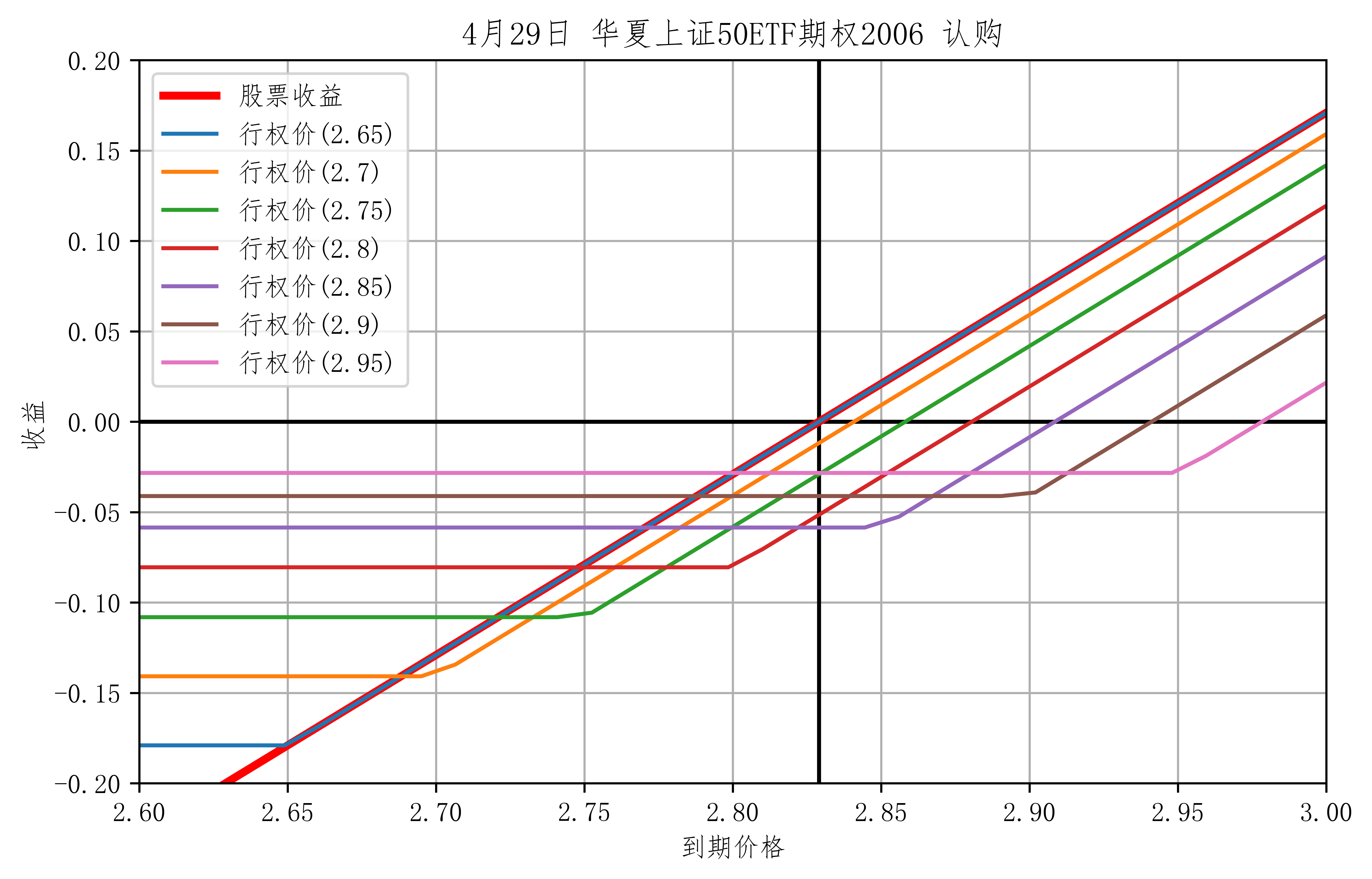• 从之前所学，可知 虚值期权 它的风险是相当高，同时当你买入实值越大的期权时，随着期权费越高，它和普通股票价格直线越接近。
2. 接下来 我们 来看看之前构造的 BlackScholes 定价系统

1. 输入当天价格 current、行权价 call_date_2006['price']、无风险利率 5%、到期时间 40/250=0.16年、默认波动率 30%
2. 对比 BS 期权价 与 实际 期权价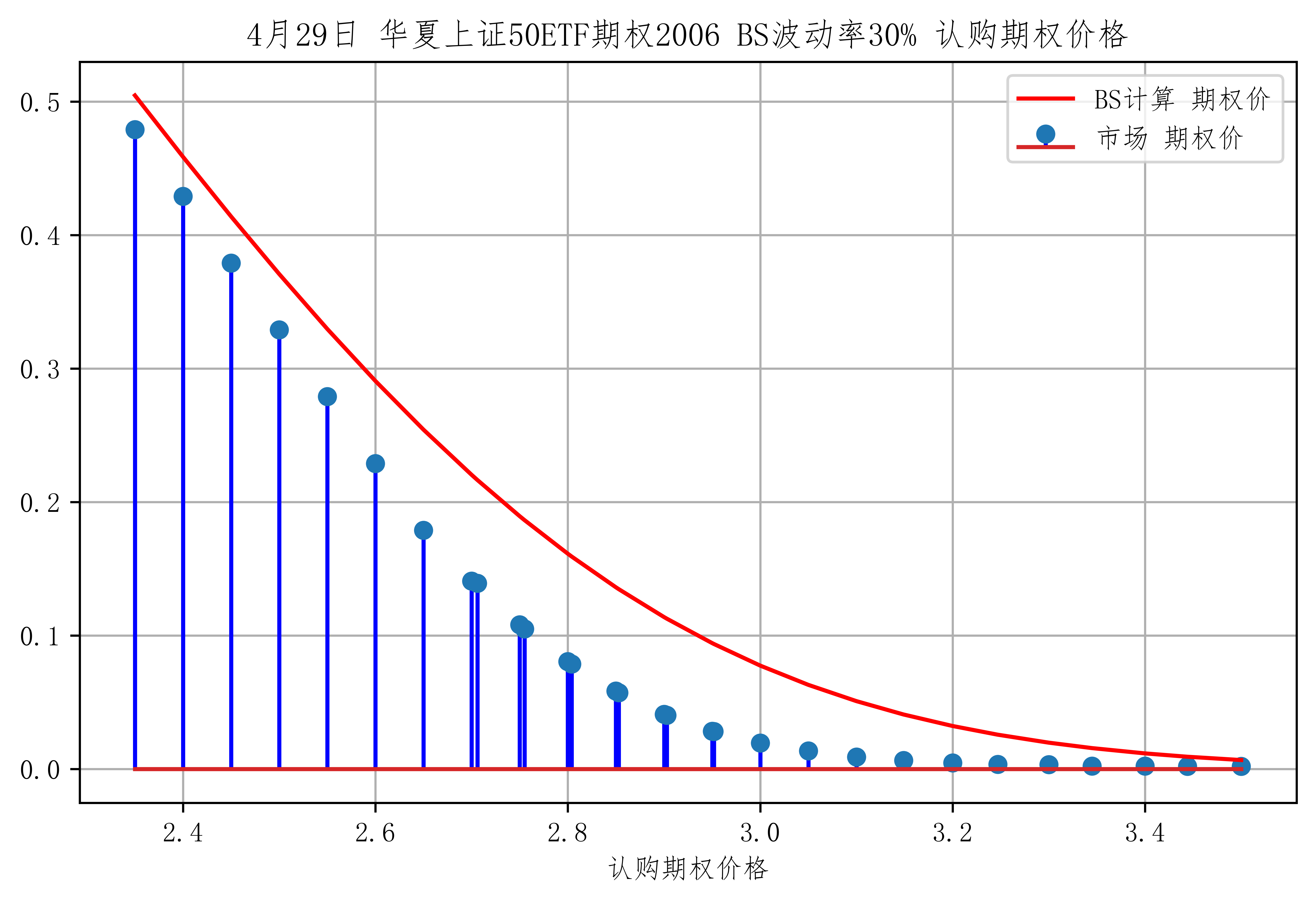1. 用 BS 求它的隐含波动率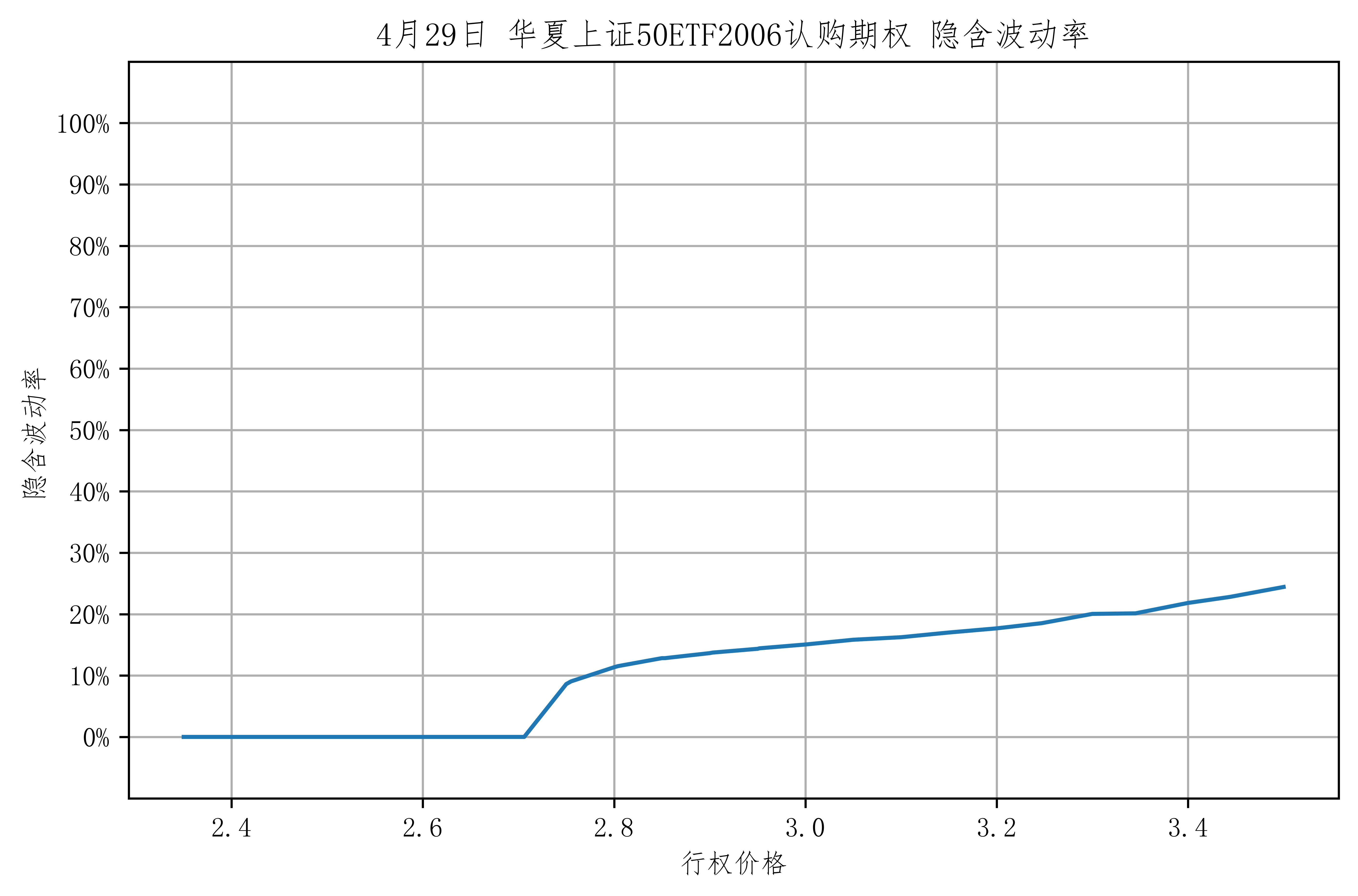### 绘制期权隐含波动率

• 结合上面的分步绘图 我们可以绘制出 并对比 当前所有可购买 50EFT 期权的隐含波动率
opt_name = pro.opt_basic(exchange='SSE')
opt_name['type']= opt_name['name'].str.extract(r'(\d+)') # 提取期权类型 300 或 50


ts_code exchange name per_unit opt_code opt_type call_put exercise_type exercise_price s_month maturity_date list_price list_date delist_date last_edate last_ddate quote_unit min_price_chg type
0 10000579.SH SSE 华夏上证50ETF期权1604认购2.15 10000.0 OP510050.SH ETF期权 C 欧式 2.15 201604 20160427 0.0412 20160225 20160427 20160427 20160428 人民币元 0.0001 50
1 10000108.SH SSE 华夏上证50ETF期权1505认购2.65 10000.0 OP510050.SH ETF期权 C 欧式 2.65 201505 20150527 0.1006 20150326 20150527 20150527 20150528 人民币元 0.0001 50
# 找到 4月 29日的期权交易数据
DATE = '20200429'

new_date = pd.merge(opt_name,opt_date,on=['ts_code'])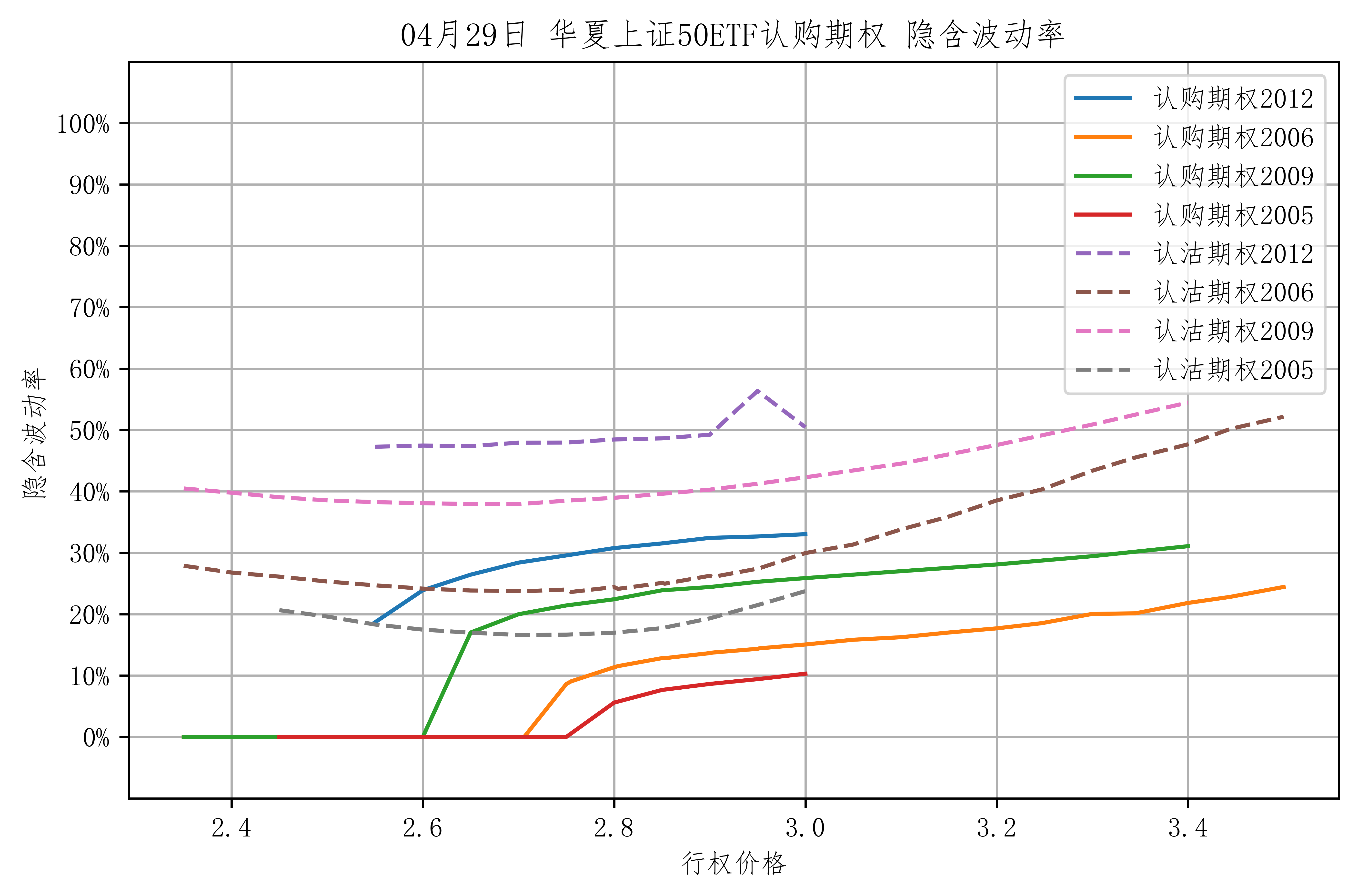• 看跌期权波动率 普遍比 看涨波动率高，说明投资者比较偏爱于购买看跌期权。

## 希腊字母

• 运用希腊字母 是对期权 比较静态的敏感分析
• 含义：其他条件不变，（某因素）变化一单位，期权价格大概变化多少？
• 标的资产价格: Delta $(\Delta)$
• 时间: Theta $(\Theta)$
• 隐含波动率: Vega $(\vartheta)$ T
• 利率: Rho $(r)$
• Gamma $(\gamma)$: 标的价格变动1单位时，Delta $\Delta$ 变多少？

### Delta $(\Delta)$ — 标的资产价格

• 标的资产价格变化一单位，期权价格大概变化多少？
• 例：
• Delta = 0.3141 意味着
• 如果指数上涨 10 点，期权价格大概上涨 3.141点
• $\Delta = \frac{\partial c}{\partial S}$
• 期权价格曲线切线斜率（动态时变）

• (无红利欧式期权) Delta 的4个特征：

#### 特征

• 特征 I

• 特征 II

• PCP 平价原理

• $看涨期权 Delta = 看跌期权 Delta + 1$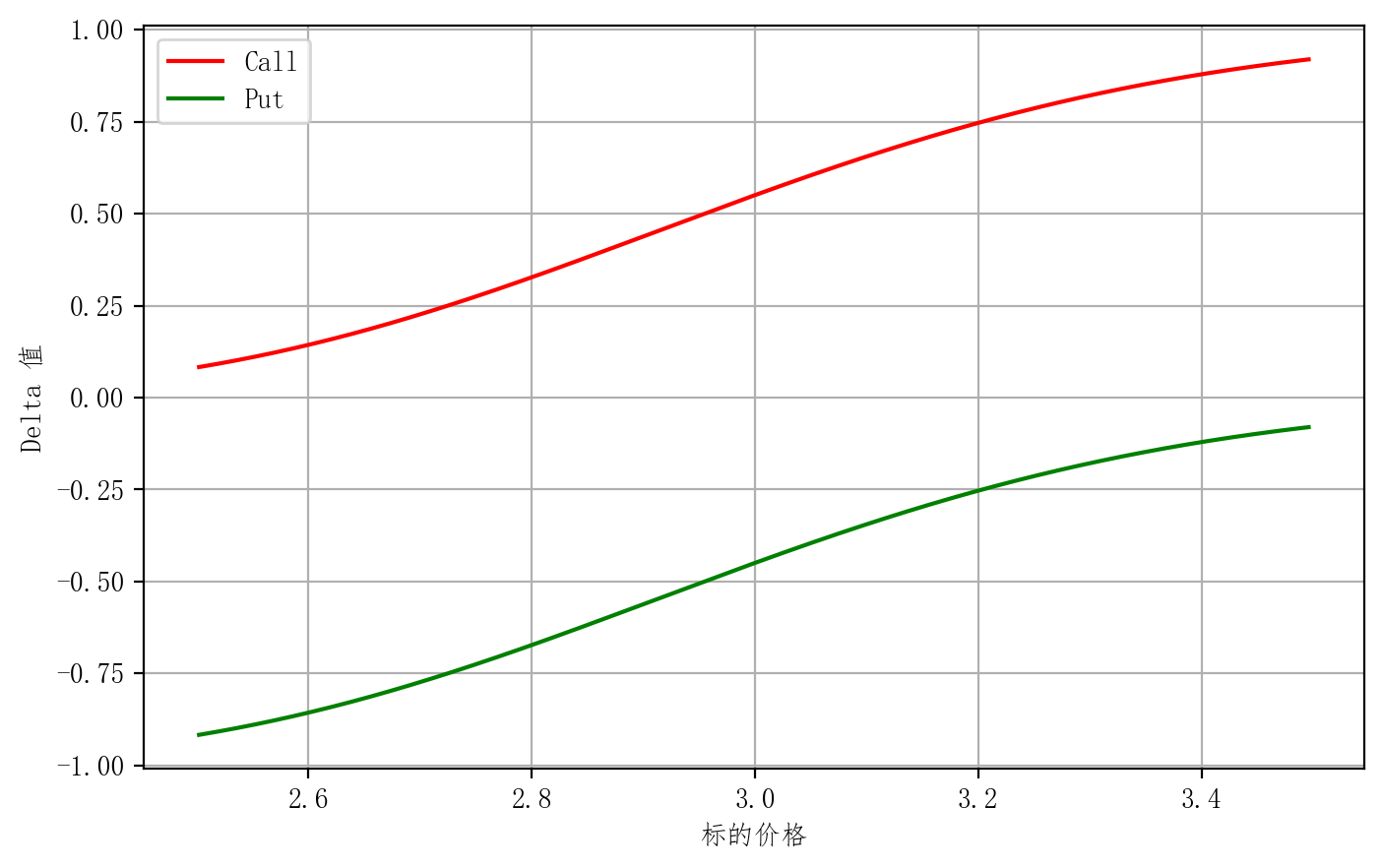• 如图，可以看出来 他们的Delta 相差1

• 特征 III

• 快到期时，实值、虚值、和平值期权的Delta 差异比较大

• 剩余期限比较大的话 时间价值比较大 它的期权价格曲线相对平滑，所以它的切线斜率比较小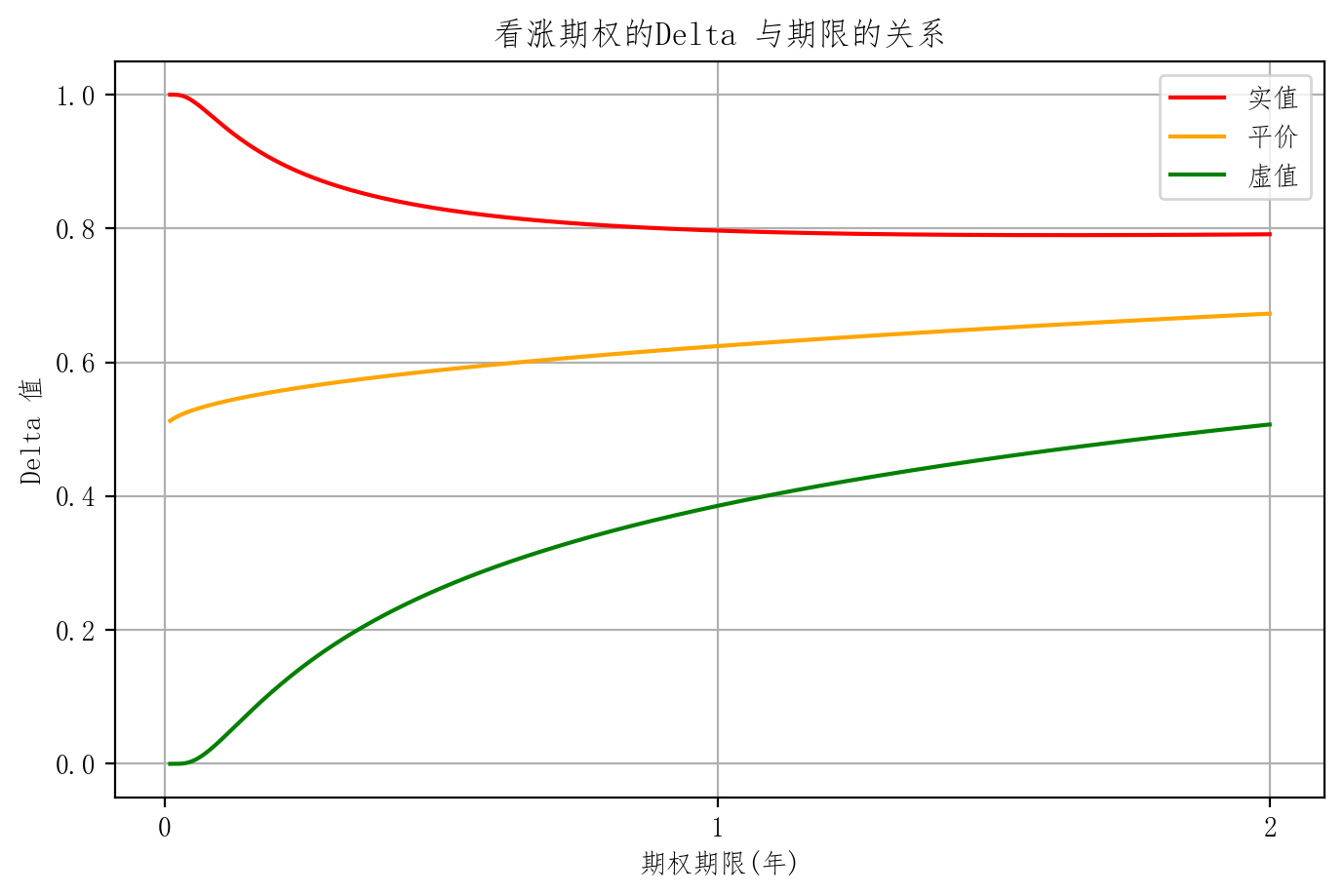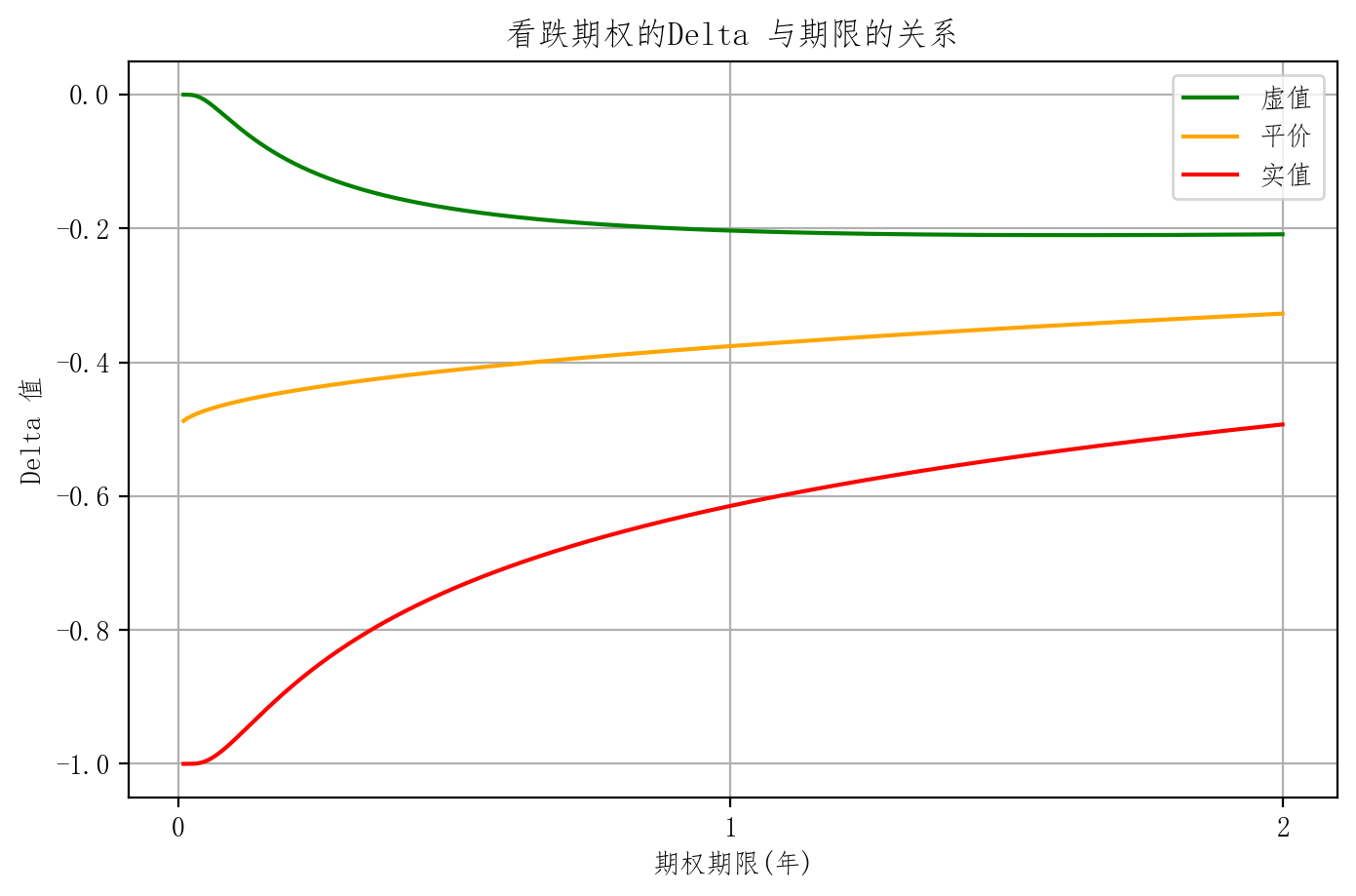• 特征 IV

• 波动率较低时，实值、虚值、和平值期权的Delta 差异比较大

• 原理与特征 III 相同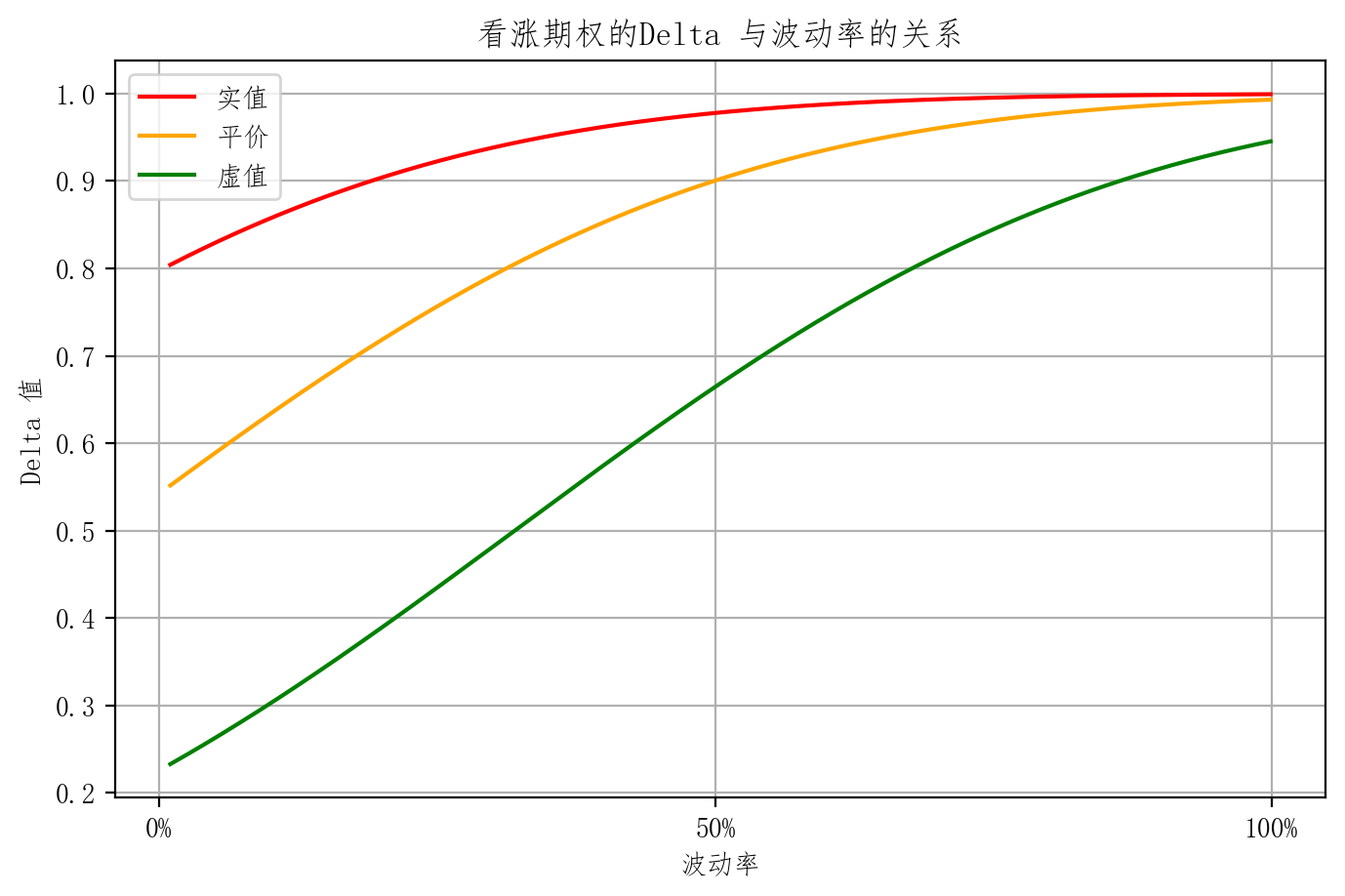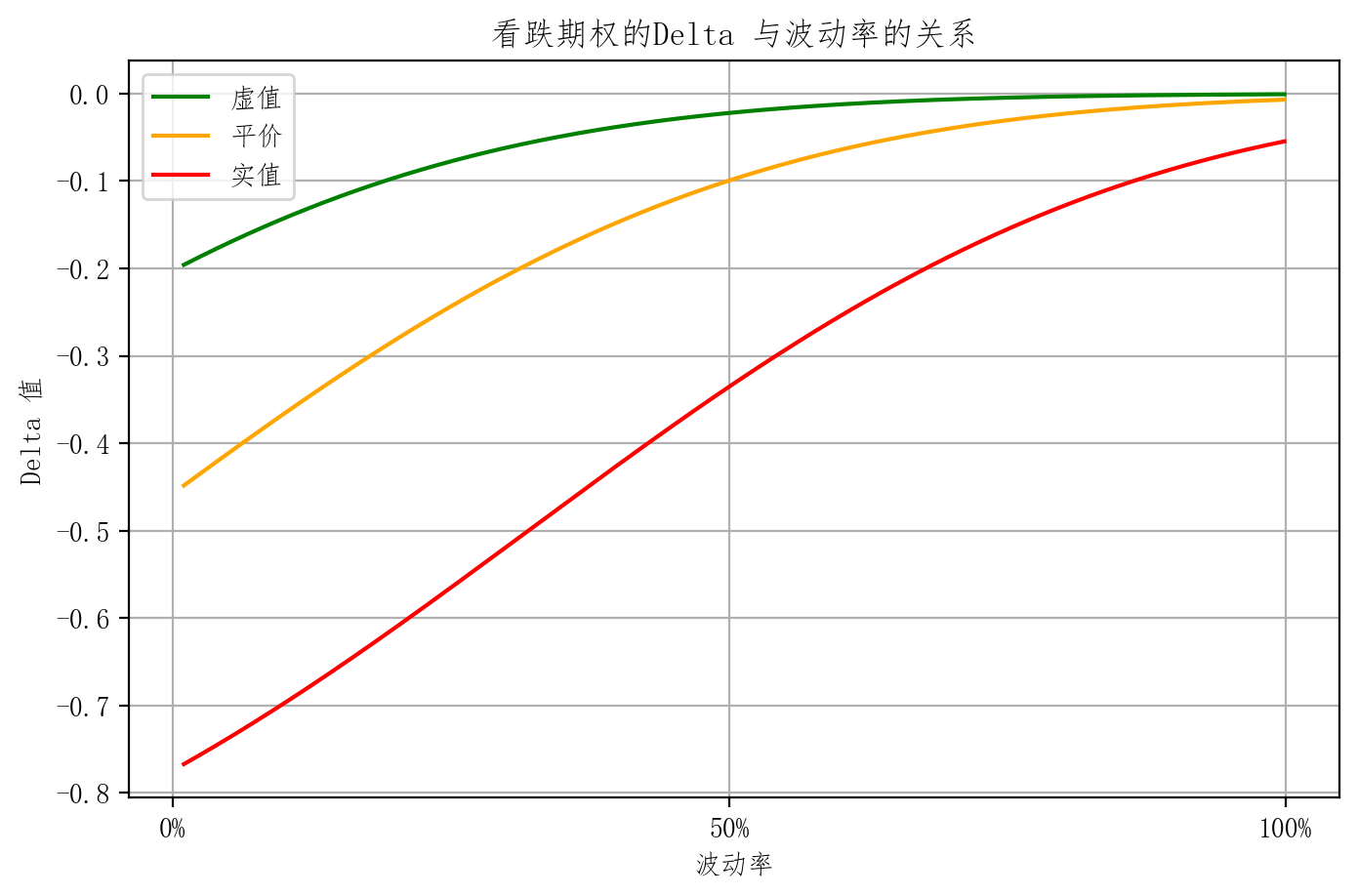#### 证券组合的 Delta 值

(无红利)
$% $ 5单位多头，每单位Delta为0.5: $5\times 0.5 =2.5$

(无红利)
$% $ 4单位空头，每单位Delta为-0.4: $4\times -0.4 =-1.6$

(无红利)
$% $ 3单位多头，每单位Delta为-0.5: $3\times -0.5 =-1.5$

(无红利)
$% $ 2单位空头，每单位Delta为0.6: $2\times 0.6 =1.2$

#### Delta 中性

• 在投资组合中 让Delta 为0 称 Delta 中性
• 意味着 投资组合对现货价格变动的一阶敏感性为 0
• 实现：运用同一标的资产的现货，期货和期权等进行相互套期保值，使证券组合的值等于0
• 特点：有期权的情况下是动态的，需要不懂调整头寸以使组合重新处于$\Delta$中性状态，这种调整称为再均衡(Rebalancing)。

### Gamma $(\Gamma)$

• 标的价格变动1单位时，Delta $\Delta$ 变多少？

• 例：
• Gamma = 0.0056，Delta = 0.3141 意味着
• 如果指数上涨 10 点，Delta大概上升至 $0.3141+10*0.0056 = 0.3701$
• 如果指数下跌 10 点，Delta大概下降至 $0.3141-10*0.0056 = 0.2581$
• $\Gamma = \frac{\partial \Delta}{\partial S}=\frac{\partial^2 c}{\partial S^2}$
• 期权价格曲线曲度的主要部分 $d c \approx \Delta \times d S + \frac{1}{2}\Gamma \times (d S)^2$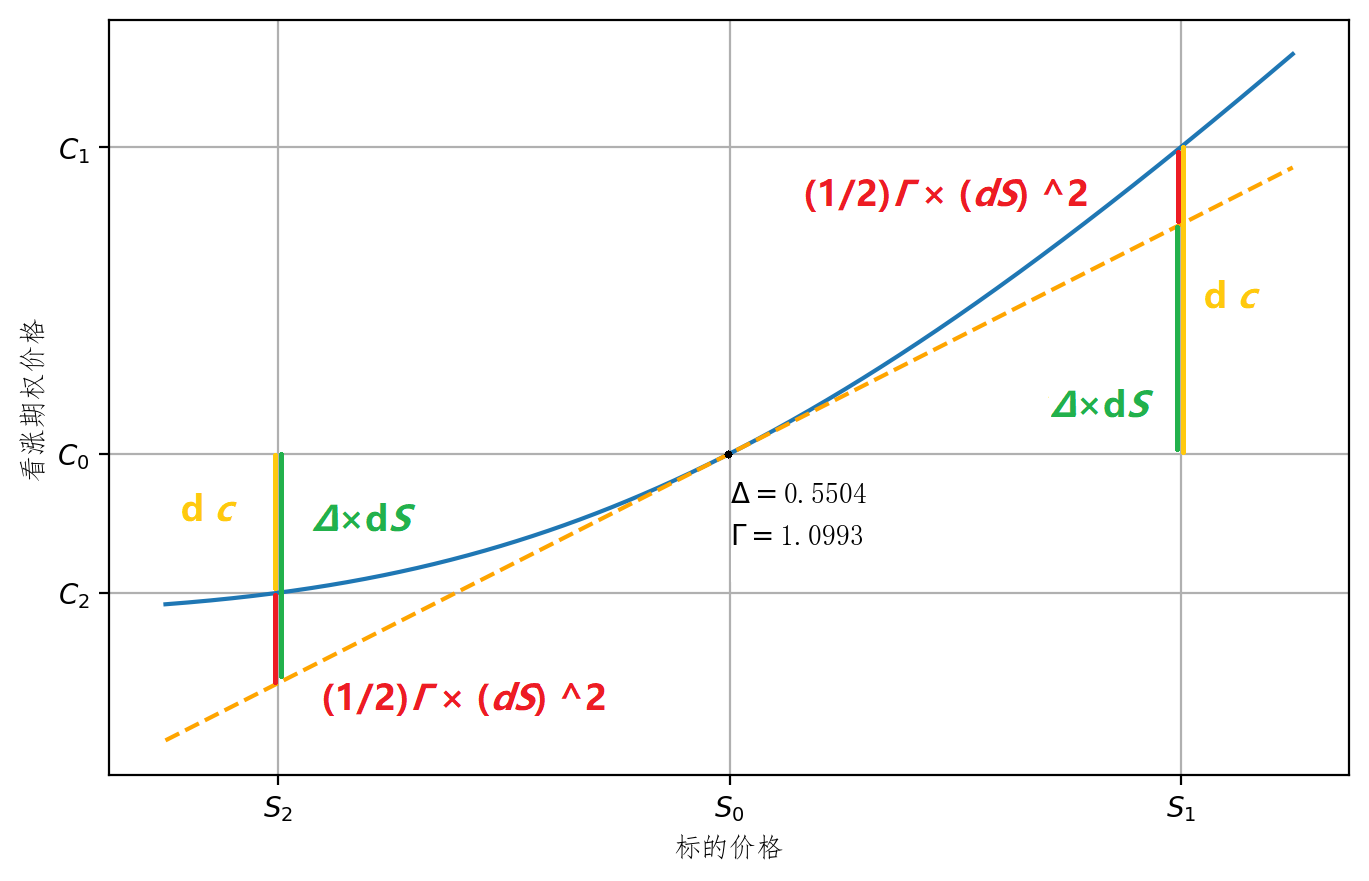• (无红利欧式期权)Gamma的4个特征

#### 特征

• 特征 I

• 期权多头 $\Gamma>0$ 凹曲面 Convex
• 看涨 Convex up 上凹
• 看跌 Convex down 下凹
• 期权空头 $\Gamma<0$ 凸曲面 Concave
• 看涨 Concave down 下凸
• 看跌 Concave up 上凸
看涨 看跌

• 特征 II

• 其他条件相同的欧式期权：看涨Gamma=看跌Gamma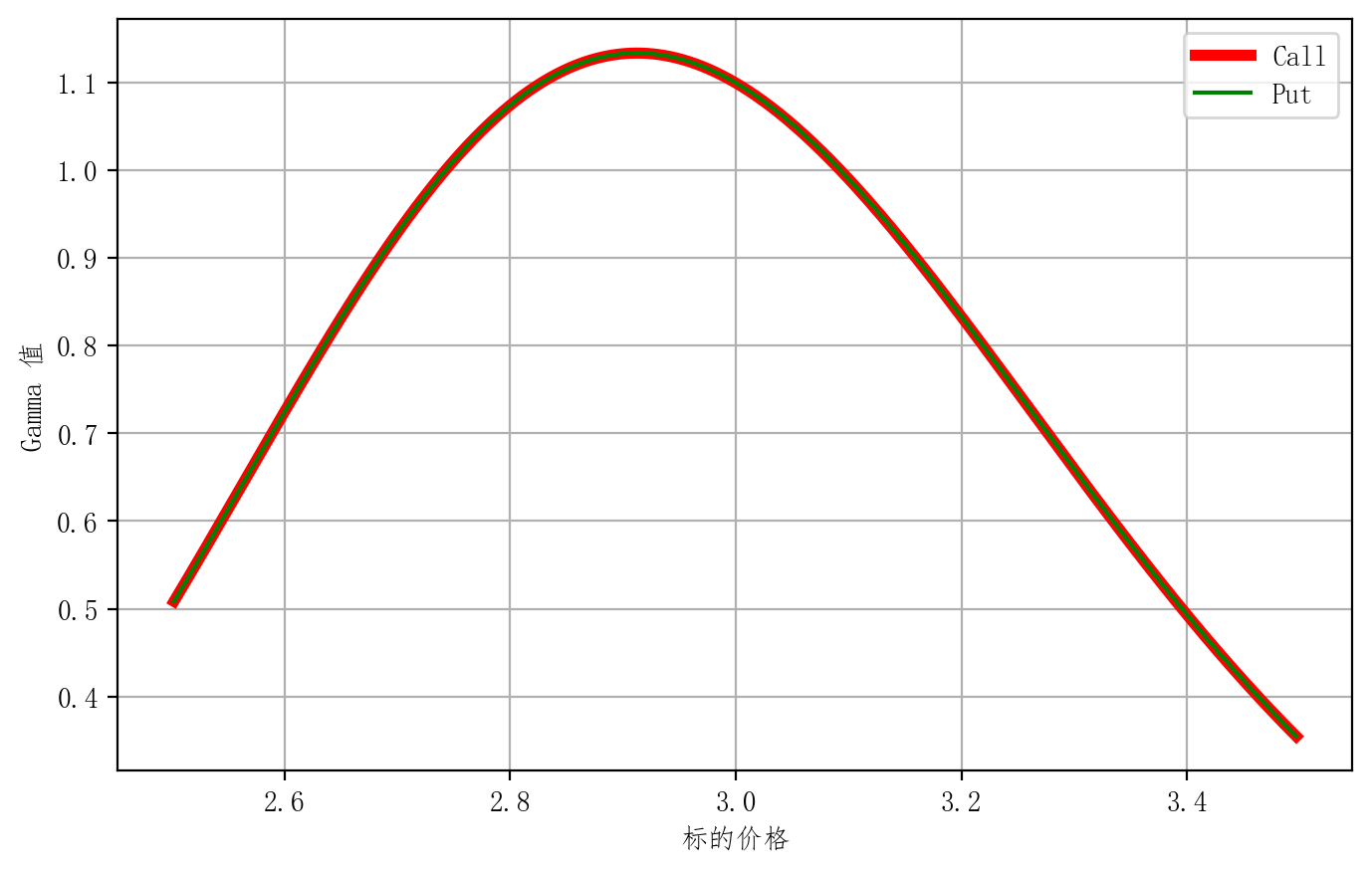• 特征 III

• 平价附近期权的Gamma值最大

• 特征 IV

• 快到期时，实值、虚值、和平值期权的Delta 差异比较大
• 波动率较低时，实值、虚值、和平值期权的Delta 差异比较大
Gamma 关系 看涨 看跌

#### 证券组合的 Gamma 值

(无红利)
$\Gamma>0$ 5单位多头，每单位 Gamma 为0.005: $5\times 0.005 =0.025$

(无红利)
$% $ 4单位空头，每单位 Gamma 为-0.004: $4\times -0.004 =-0.016$

(无红利)
$\Gamma>0$ 3单位多头，每单位 Gamma 为0.005: $3\times 0.005 =0.015$

(无红利)
$% $ 2单位空头，每单位 Gamma 为-0.006: $2\times -0.006 =0.012$

#### Gamma 中性

• 只有期权有 Gamma 值
• 在投资组合中 让 $\Gamma$ 为0 称 $\Gamma$ 中性
• $\Gamma$ 中性 时为了消除 $\Delta$ 中性的误差，同样也是动态的概念
• 实现：由于保持 $\Gamma$ 中性只能通过期权头寸调整获得，实现中性的结果往往时非中性，因而常常还需要运用标的资产或期货头寸进行调整，才能使得证券组合同时实现 $\Delta ,\ \Gamma$ 中性

### Theta $(\Theta)$ — 时间

• 时间变化一天，期权价格大概变化多少？
• Time 时间

• 例：
• Theta = 1.234 意味着
• 时间每过一天，期权价格大概上涨 1.234点
• $\Theta = \frac{\partial c}{\partial t}$

#### 特征

• 特征 I

• 期权的 $\Theta$ 通常为负：一般来说，随着到期日的临近，期权的价格逐渐衰减 (time decay)
• 处于 深度实值状态的无红利资产欧式看跌期权和处于实值状态的标的资产红利很高的欧式看涨期权，$\Theta$ 可能为正

• 特征 II

• 剩余期限越短, (time decay) 速度越快, $\Theta$ 负得越多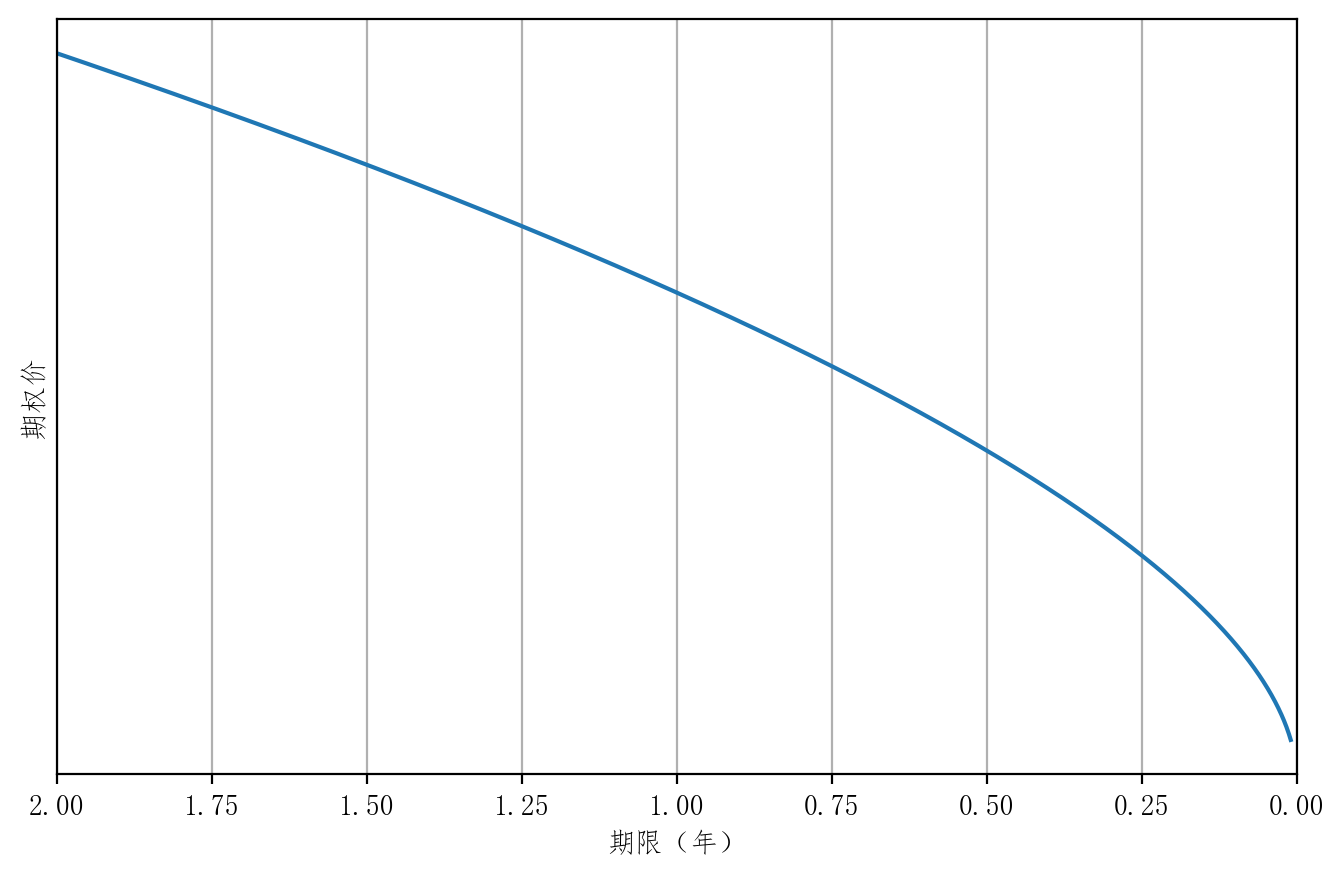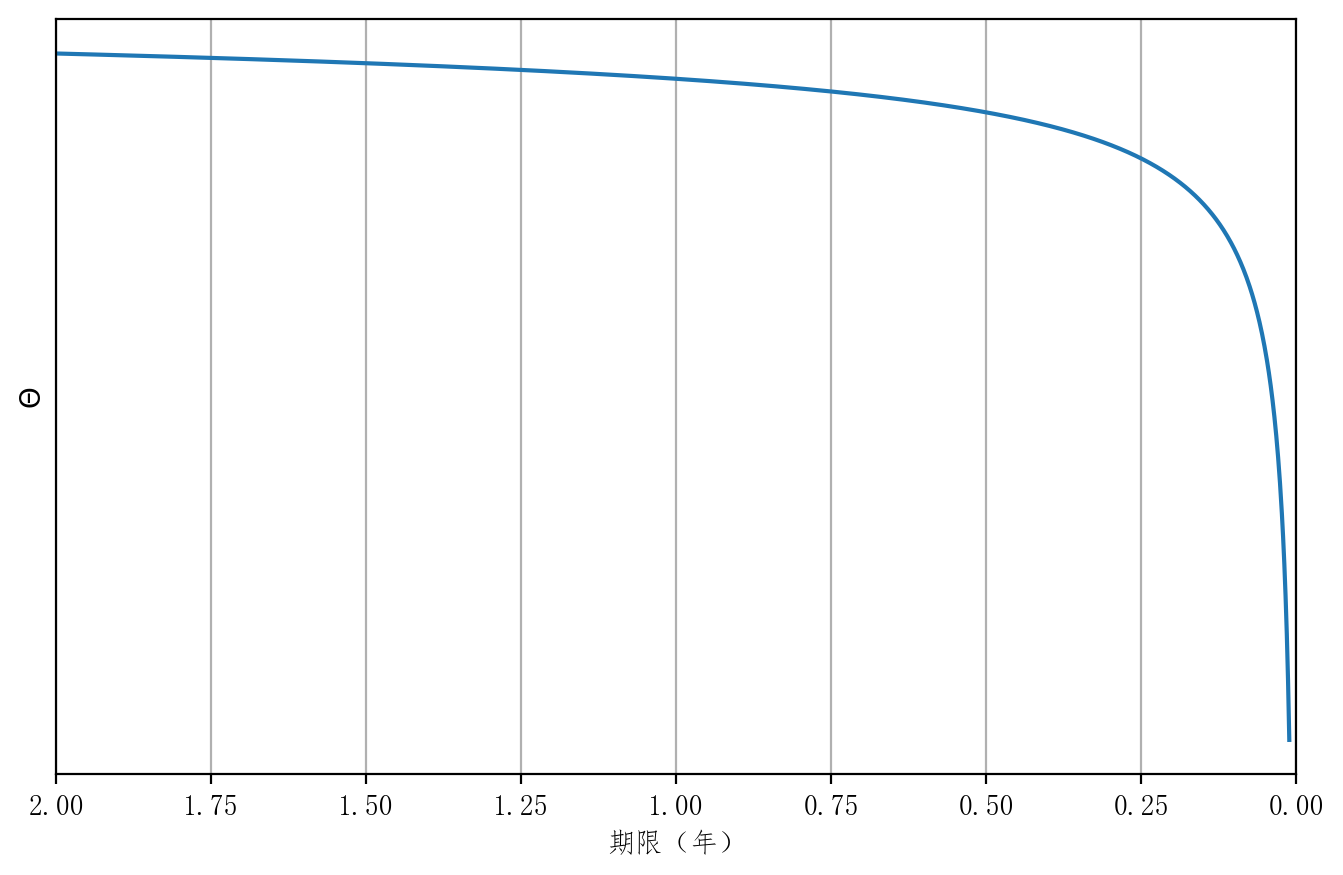• 特征 III

• 与实值、虚值期权相比，平价期权Theta 负值最大

• Theta 与标的资产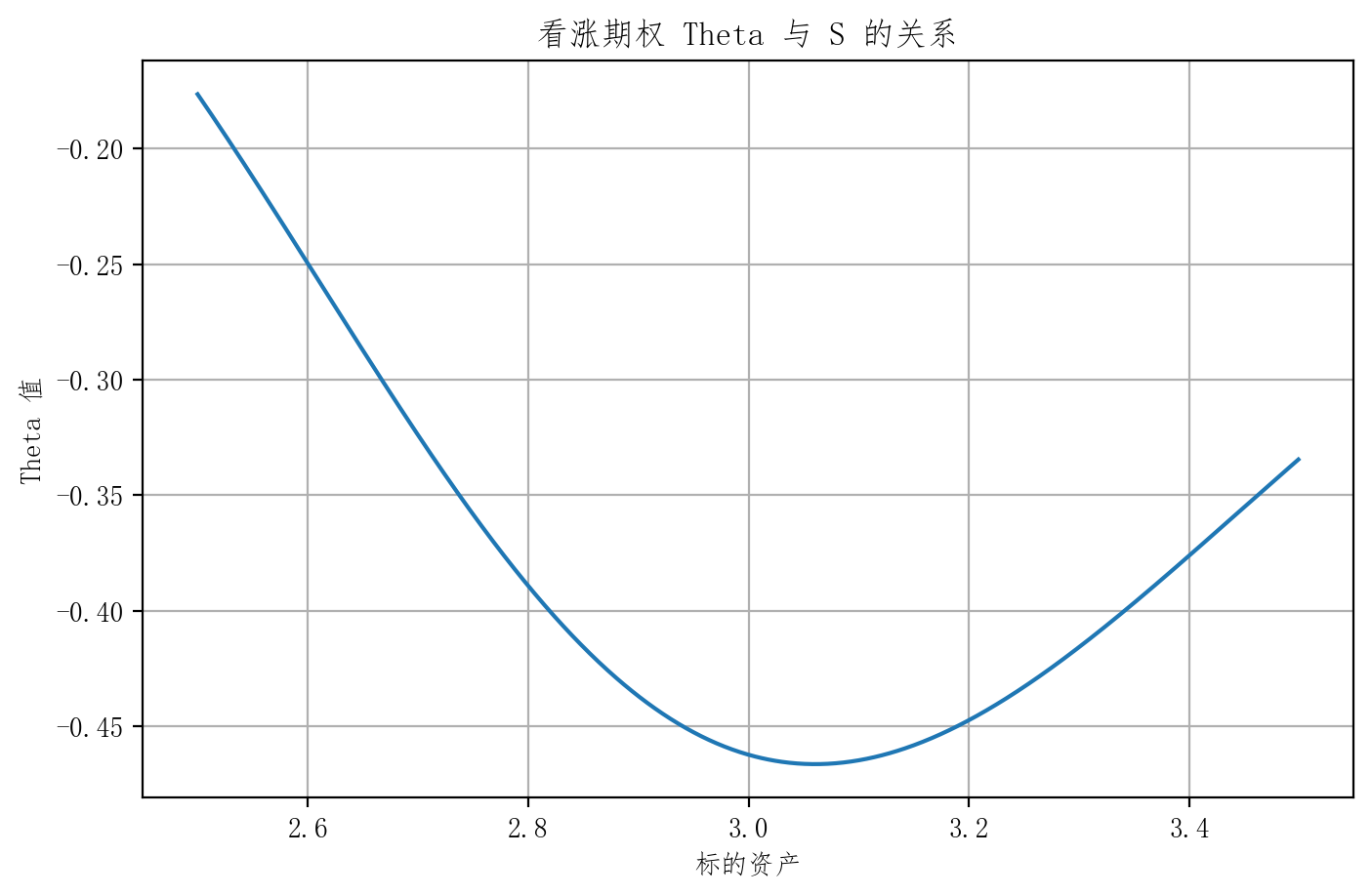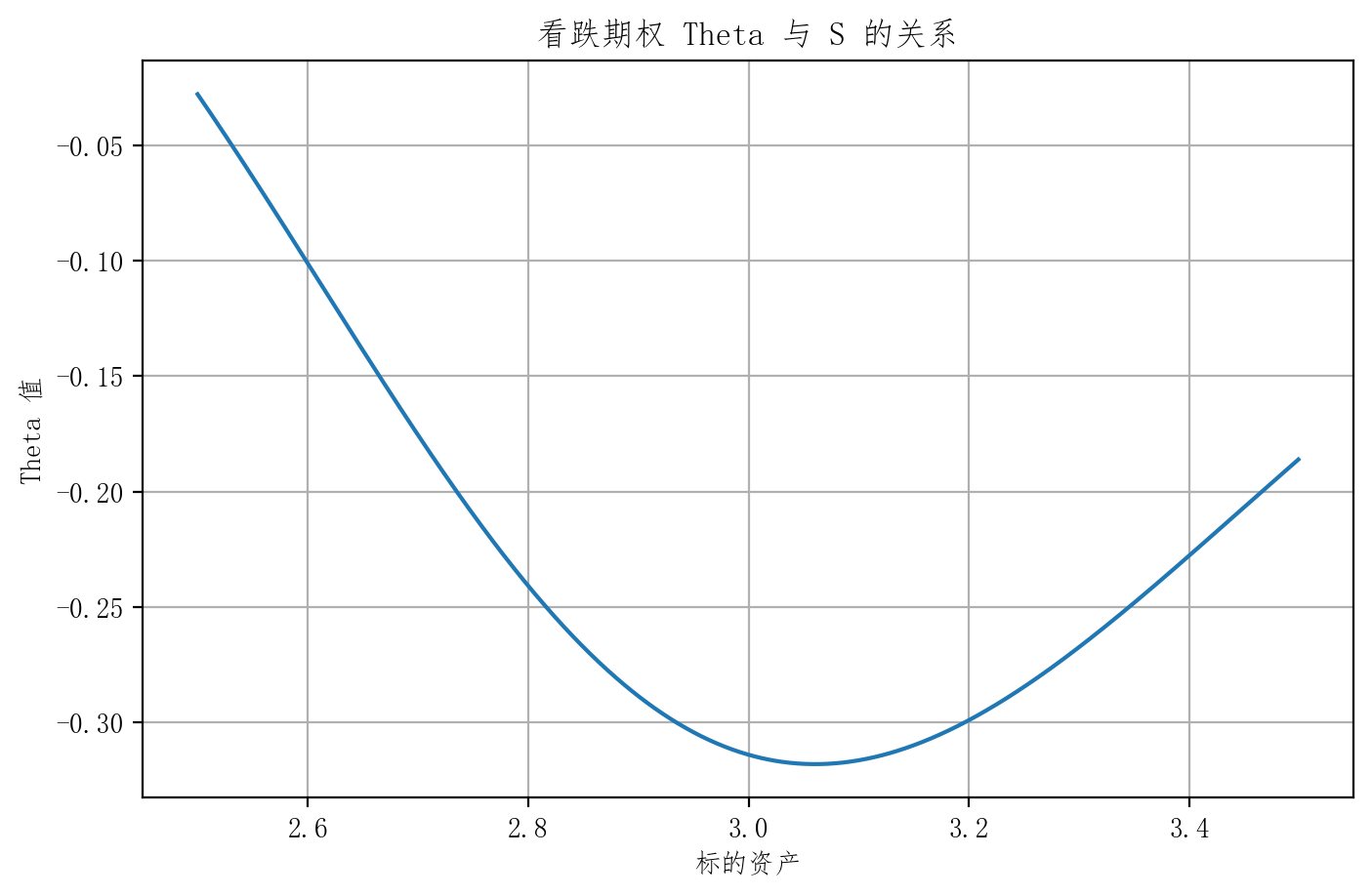• 特征 IV

• 快到期时，实值、虚值和平价期权Theta 差异最大
• 越快到期 平值的敏感性越高
• 看涨Theta与期限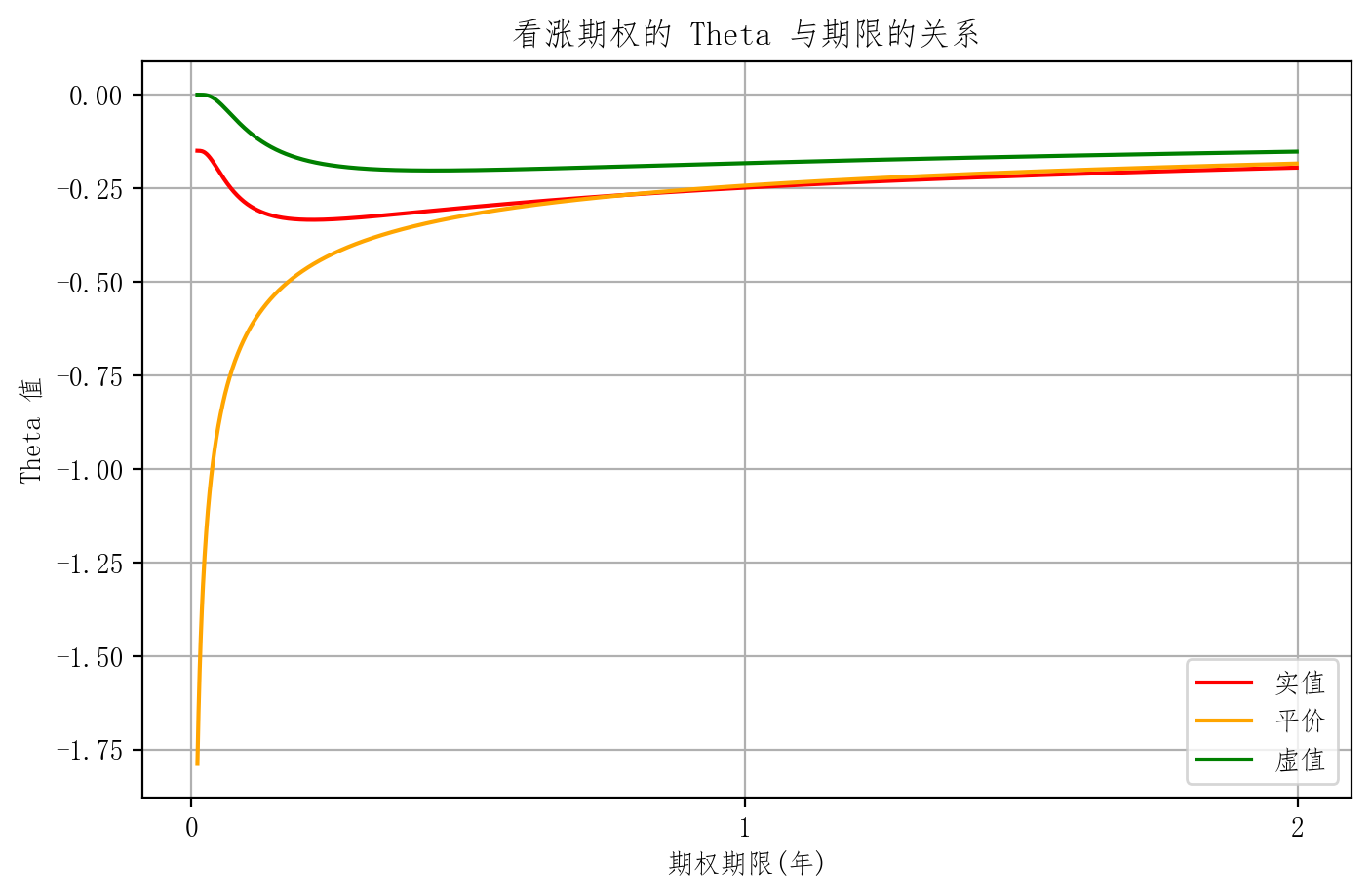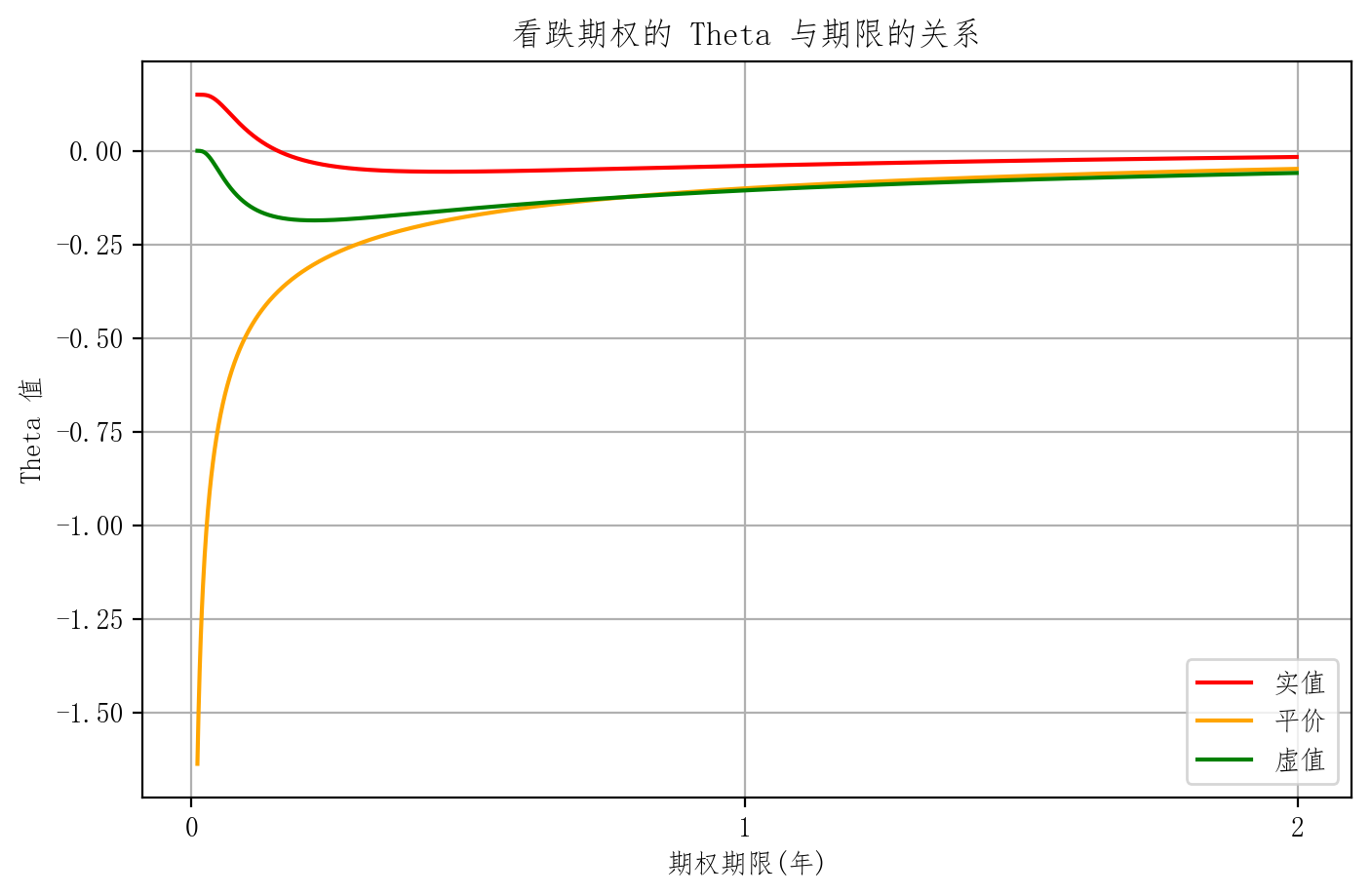• 时间推移时确定的，没有风险可言，因此无需 Theta 中性
• Theta 值大小反映了期权购买者随时间推移所损失的价值，因而Theta 值仍是一个重要的敏感性指标

### Vega $(\varsigma)$ — 隐含波动率

• 隐含波动率变化一百分点，期权价格大概变化多少？
• Volatility 隐含波动率

• 例：
• Vega = 1.878 意味着
• 每隐含波动率上升1%，期权价格大概上涨 1.878点
• $\varsigma =\frac{\partial c}{\partial \sigma}$

#### 特征

• Vega >0
• (欧式)看涨 Vega = 看跌 Vega $c + \frac{X}{1+r(T-t)}=p+S \to \frac{\partial c}{\partial \sigma}= \frac{\partial p}{\partial \sigma}$
• 平价期权的 Vega 较大
• 剩余期限越长，Vega 越大
• 例 同样年化波动率 16% 的1年与2年期权 他们的波动率不一样：一年 $16\%$ 两年 $16\%\times \sqrt{2}$

#### 证券组合的 Vega 值

(无红利)
$\Gamma>0$

(无红利)
$% $

(无红利)
$\Gamma>0$

(无红利)
$% $

#### Vega 中性

• 只有期权有 Vega 值
• 证券组合 Vega 值 为零时 称为处于 Vega 中性状态
• Vega 中性是为了消除 隐含波动率变化 的影响，同时也是动态的概念
• 由于保持 Vega 中性 只能通过期权头寸调整获得，实现 Vega 中性的结果往往是 $\Delta$ 非中性 和 $\Gamma$ 非中性，因而常常还需要运用标的资产、期货头寸、期权头寸进行调整，才能使得组合同时实现 $\Delta$ 中性、$\Gamma$中性和 Vega 中性

• Rate 利率

## 完

• 总算是在假期把它写完了，错误应该还是蛮多的，欢迎在评论区留言。
• 金融类 之后有时间和兴趣的话 应该还会写联系 Machine Learning 做股票预测之类的文章。
• 如果有帮助的话 请给我一键三连 ~ ~ ~

Support